• 总结一下平时可能会用到的工具性网站，欢迎补充。

### 概览

地址 描述
Regex101 强大的正则表达式工具
Compressor.io 强大的图片在线压缩工具
TinyPNG 强大的图片在线压缩工具
VisuAlgo 各种算法可视化
Desmos Graphing Calculator 在线数学作图工具
Math 在线计算器，可以识别手写
Latex2png 将 Latex 转换为漂亮图片
Smallpdf PDF 转换操作
ASCIIFlow Infinity Ascii 作图工具
CloudConvert 强大的格式转换网站
Mathcha 数学表达式在线编辑工具
TableConvert 玩转表格
BibLaTeX Linter 在线验证你的 BibLaTeX 文件
PDF Compressor 压缩 PDF 文件
Free Online OCR OCR，识别图片文字

### Regex101 - online regex editor and debugger

强大的正则表达式工具，你可以实时查看匹配信息，并且会用不同的颜色将 Group 标记出来。而且有 Quick Reference 来帮助你记忆正则表达式的规则和 Explanation 对你的正则表达式进行解释。重要的是，支持直接生成多种语言的代码。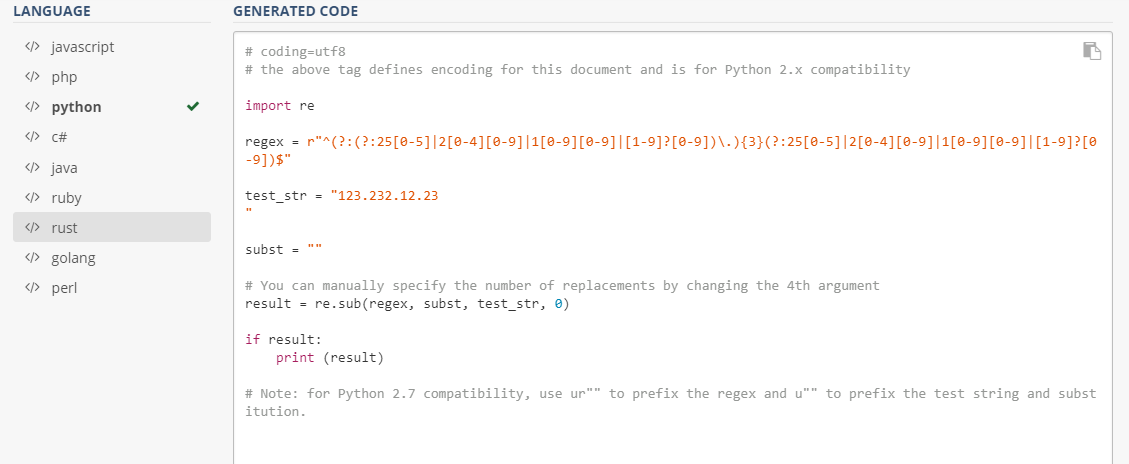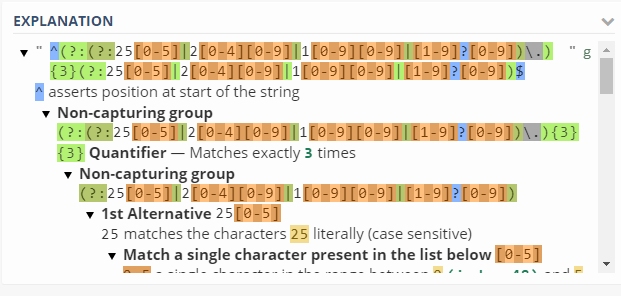### Compressor.io（需要翻墙）

强大的图片在线压缩工具，支持JPEG、PNG、GIF、SVG，在保证超高压缩率的同时保证肉眼看不出与原图的差别。（下图：1.07 MB 的图片压缩变成171.41 KB，而视觉效果几乎没有变化。）### TinyPNG

同样是图片压缩工具，虽然只支持JPEG、PNG，但是这个不需要翻墙，效果也非常棒。### VisuAlgo（多语言支持）

各种算法可视化，我的多动态图详细讲解二叉搜索树 用的动态图片就是用它做的。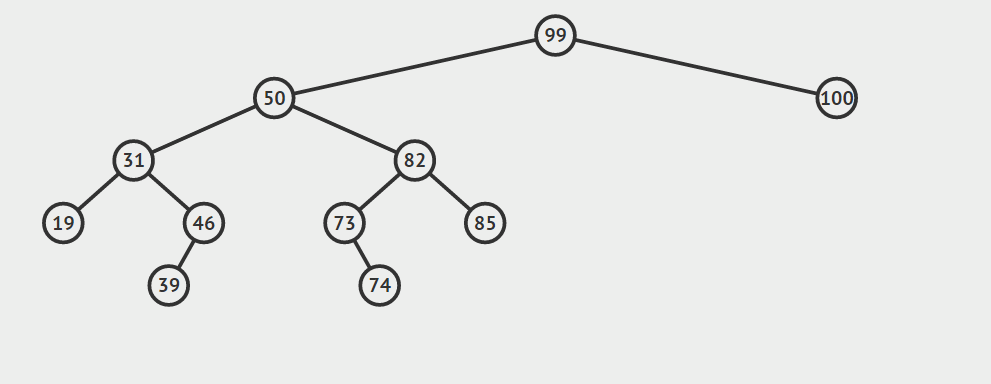### Desmos Graphing Calculator

非常强大的在线作图工具，而且支持动画（支持函数动态变化），界面也非常简洁耐看，我的循环神经网络（Recurrent Neural Network）简介 最后的图片就是用它做的。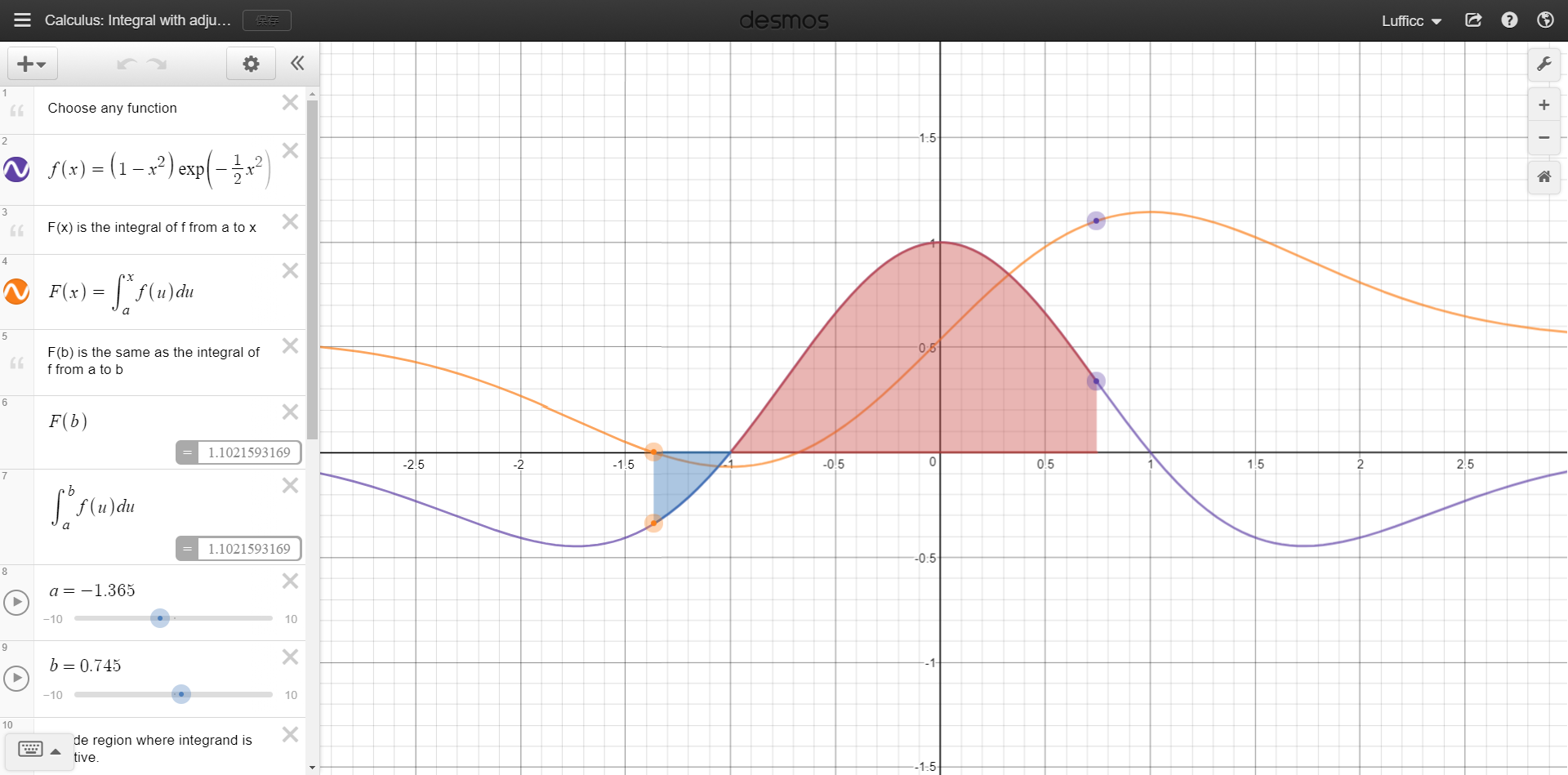### Math

在线计算器，可以识别手写的复杂表达式，而且画出函数图像，支持导出为Latex、MathML、SymbolTree等格式。### Latex2png

将 Latex 表达式转换为漂亮的、透明的 PNG 图片，很实用的网站。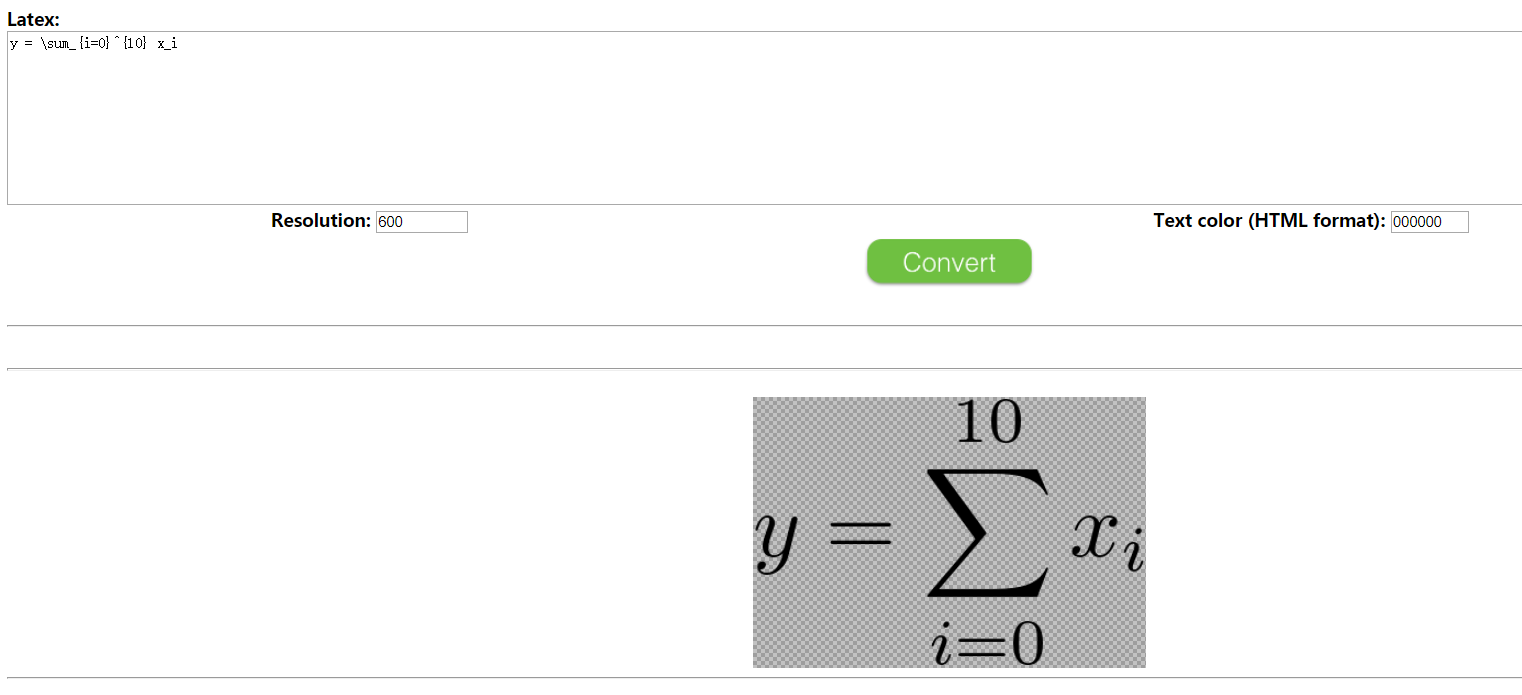### Smallpdf

和 PDF 转换有关的操作它都有，而且非常准确。支持哪些？看图片（多语言支持）：### ASCIIFlow Infinity

Ascii 作图工具，很强大。

``````+--------+                               +---------------+
|        +-+(A)+ Authorization Request +^+   Resource    |
|        |                               |     Owner     |
|        +^+(B)++ Authorization Grant +--+               |
|        |                               +---------------+
|        |
|        |                               +---------------+
|        +-+(C)++ Authorization Grant +-^+ Authorization |
| Client |                               |     Ser^er    |
|        |                               +---------------+
|        |
|        |                               +---------------+
|        |                               |     Ser^er    |
|        +^+(F)+-+ Protected Resource +--+               |
+--------+                               +---------------+

``````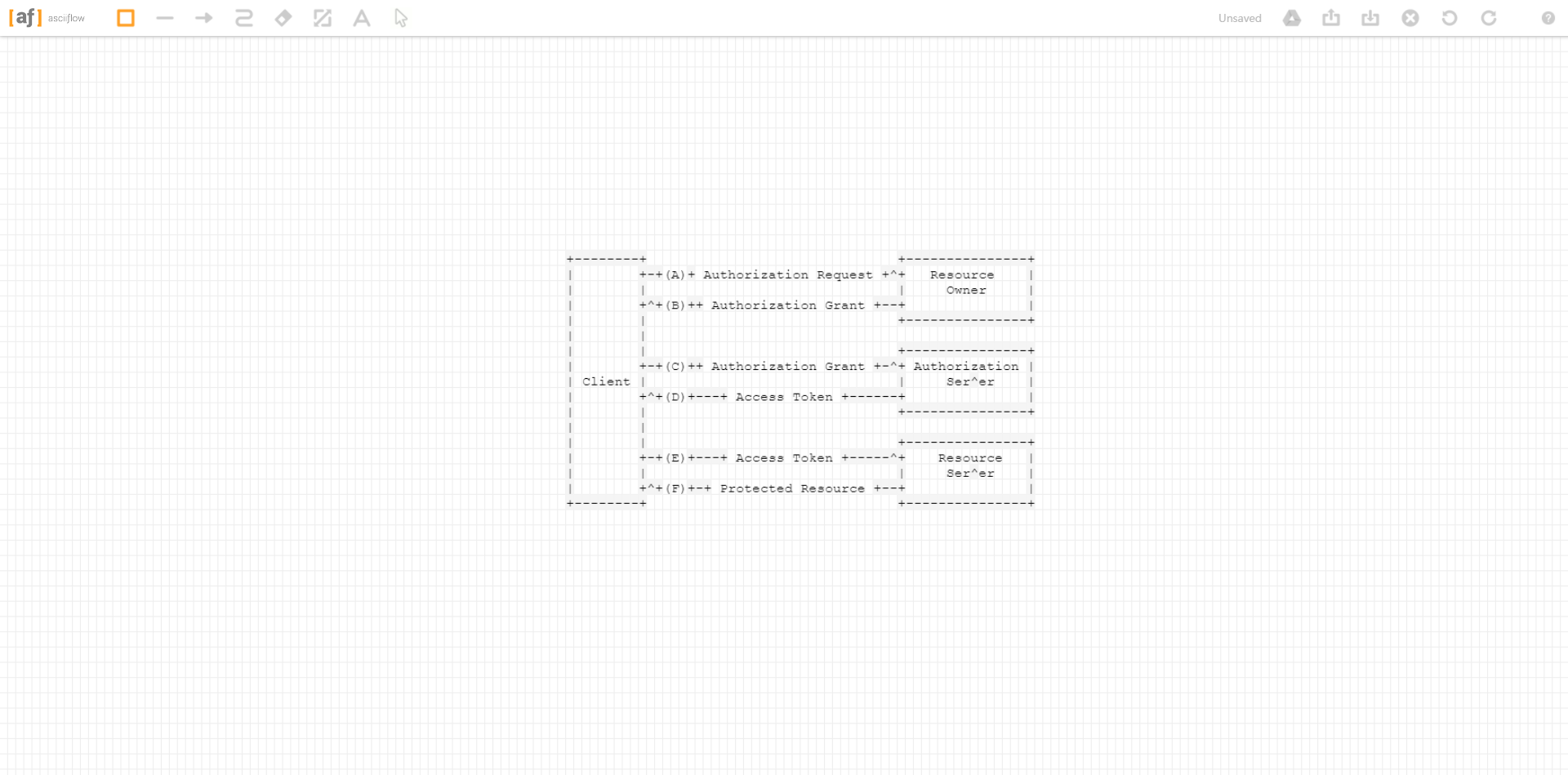### CloudConvert - Conversion Types

又一个格式转换网站，太强大了，你能想到的格式转换几乎都有：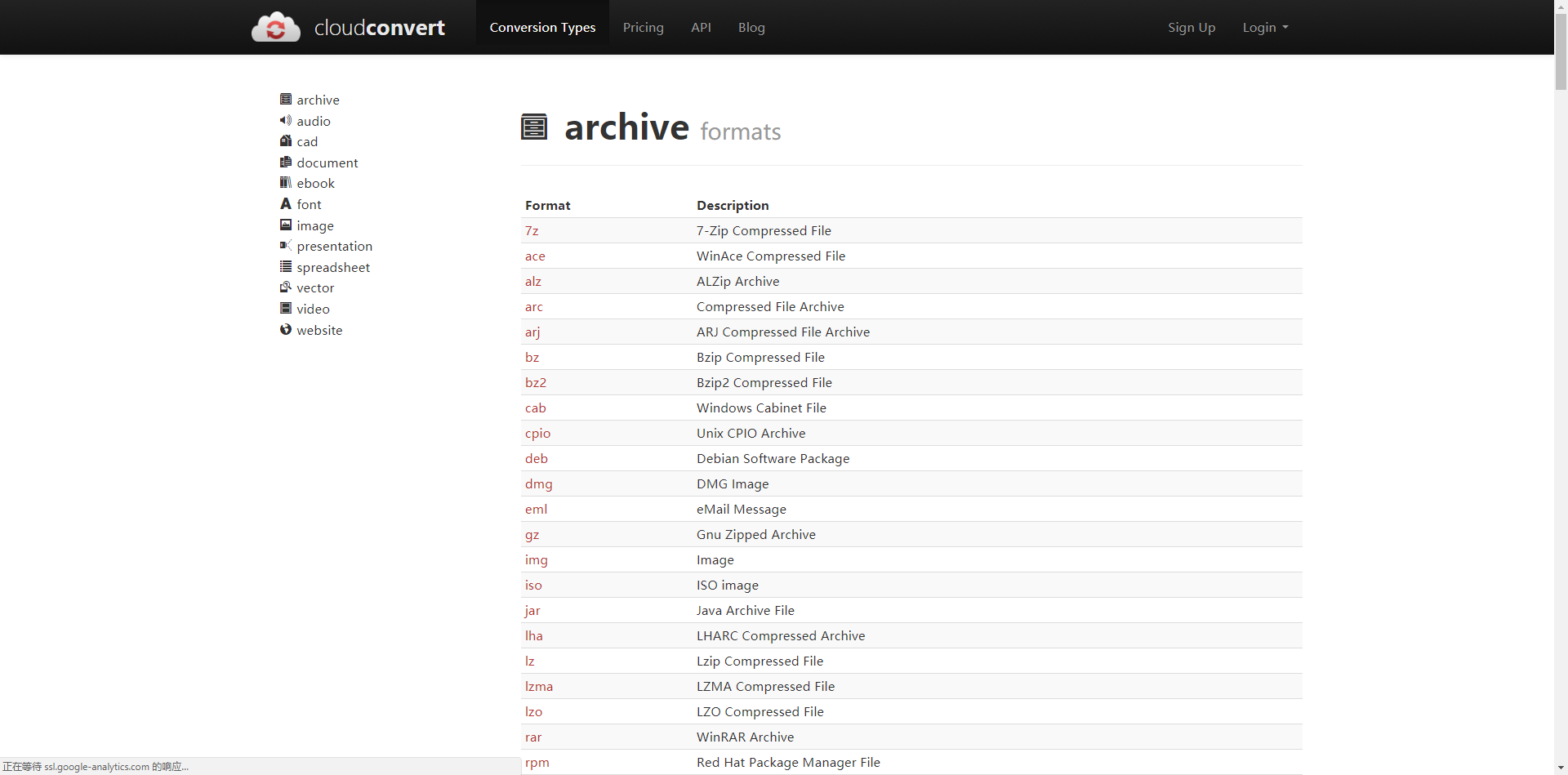### Mathcha

强大的数学表达式在线编辑工具，可以导出为PDF、Latex等格式。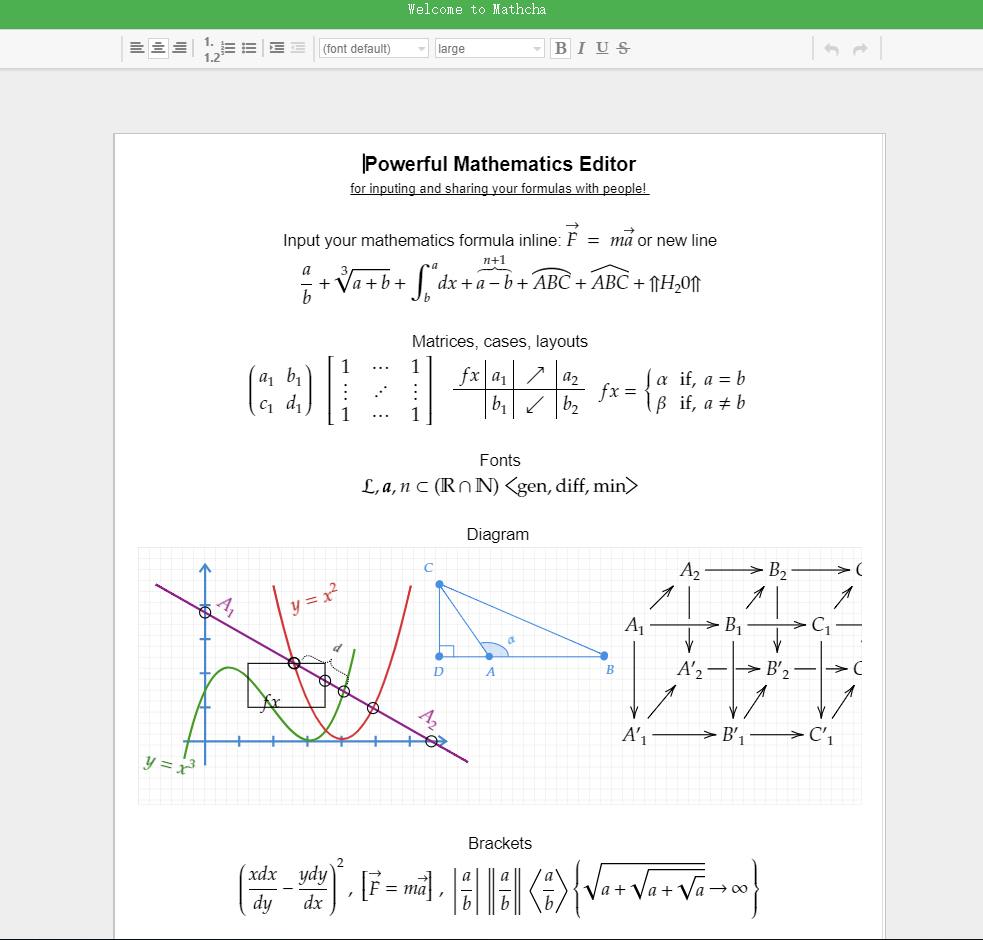### TableConvert

玩转表格，能将 Excel, URL, HTML, JSON, CSV, Markdown 和 LaTex 表格转换成 CSV/TSV, XML, YAML, Markdown table, insert SQL, HTML, Excel 和 LaTeX 格式表格。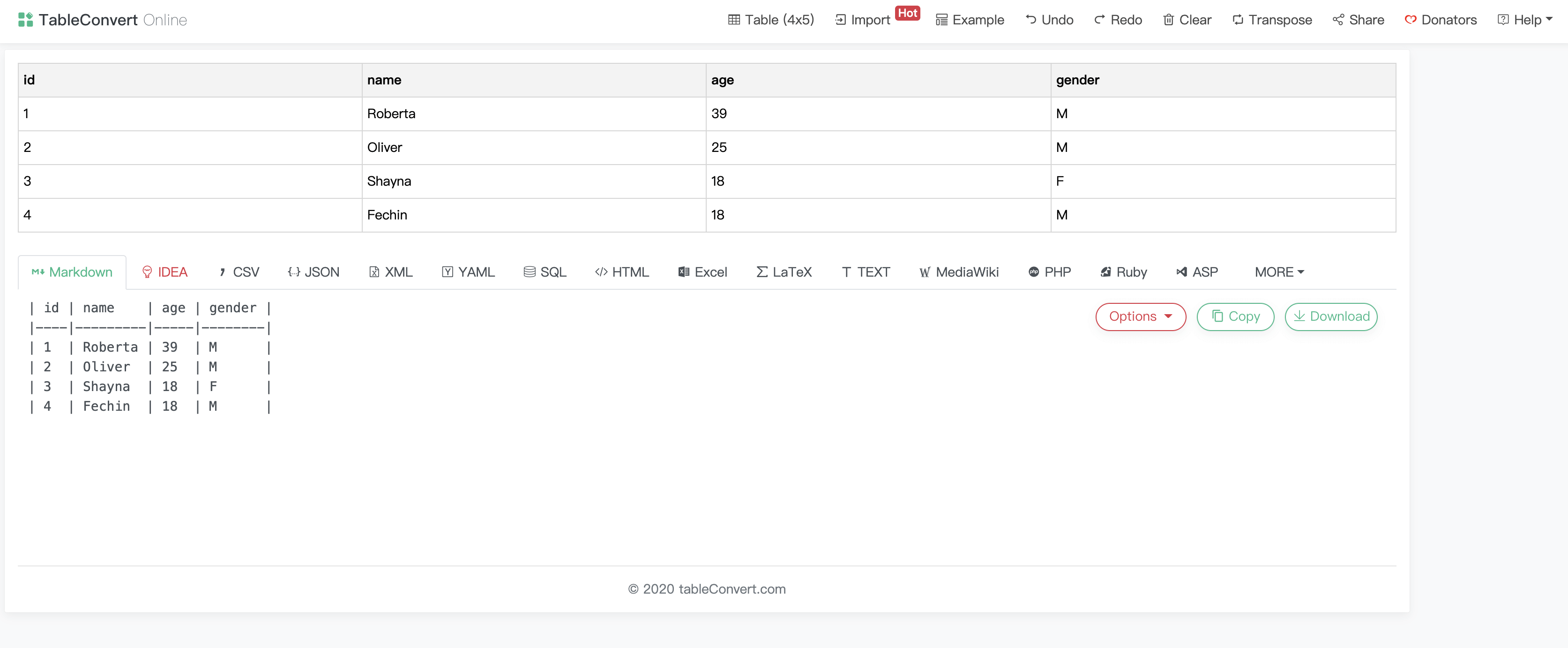### BibLaTeX Linter

在线验证你的 BibLaTeX 文件。遍历参考文献列表，并检查某些必填字段是否可用，例如，是否为每个出版物指定了年份，或者期刊文章是否具有卷号和发行号。

### PDF Compressor

压缩 PDF 文件且不会改变 DPI，并保证文档可打印、缩放。### Free Online OCR

免费的在线OCR（光学字符识别）服务，可以分析您上载的任何图像文件中的文本，然后将图像中的文本转换为可以在计算机上轻松编辑的文本。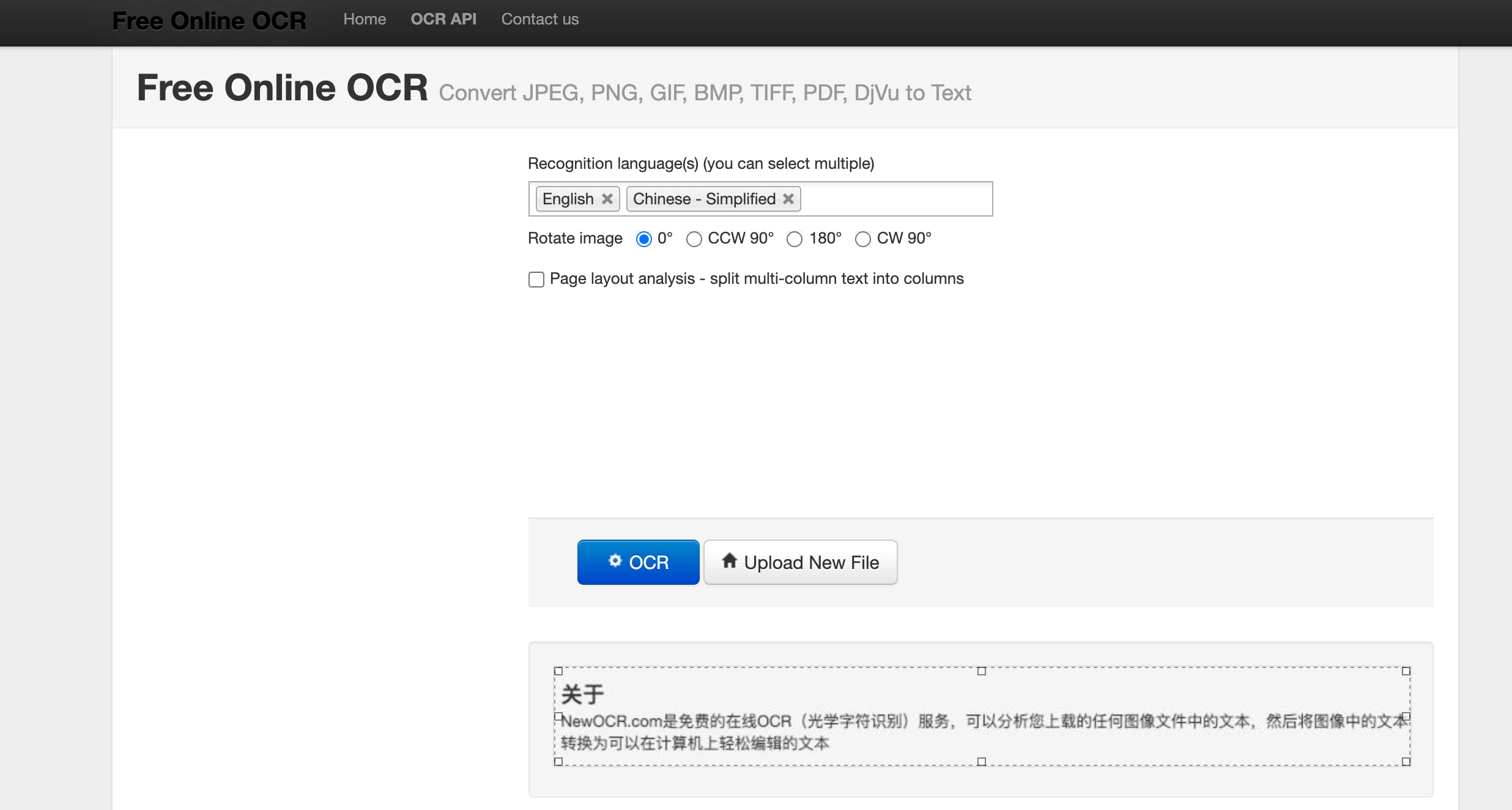• 我们人类理解事物都是基于上下文的，一句话只有在明确的上下文中才能有确定含义。

而传统的神经网络不能实现，因为神经网络是一对一的，我们输入一个 `input`，网络输出一个 `output`，而多个 `input` 之间却没有联系。就好比我们我们输入一部电影的一帧，让它预测接下来将会发生什么，显然没有先前剧情的支撑神经网络不可能做出好的预测。

## RNN

Recurrent neural networks 正是为了解决此类问题诞生的。RNN 网络连接中存在循环，并且能够存储信息。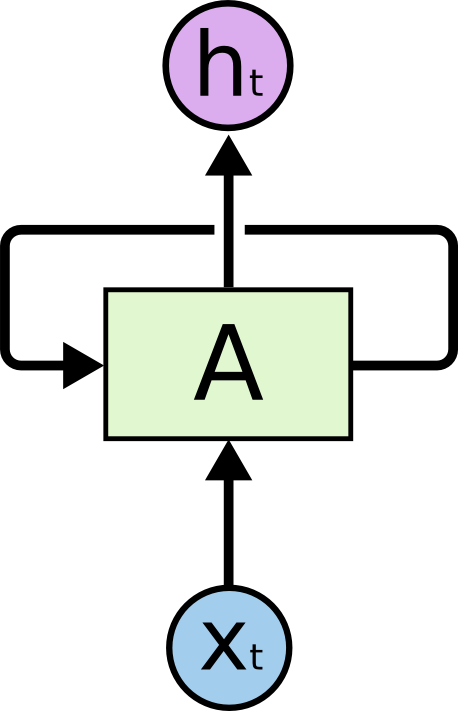上图就是一个 RNN，输入 `xt`，得到 `ht`。区别在于 A 存在循环，允许将上一步的结果传递给下一步。如果不考虑循环，和普通的神经网络没有区别，都是给予输入，得到输出。但是循环的存在，可以让上次的运算结果传递给下一次使用，这样在携带了上次的“记忆”之后，本次运算会在上次的运算基础上做出更好地预测。如果我们把每一步展开来看，会更简单：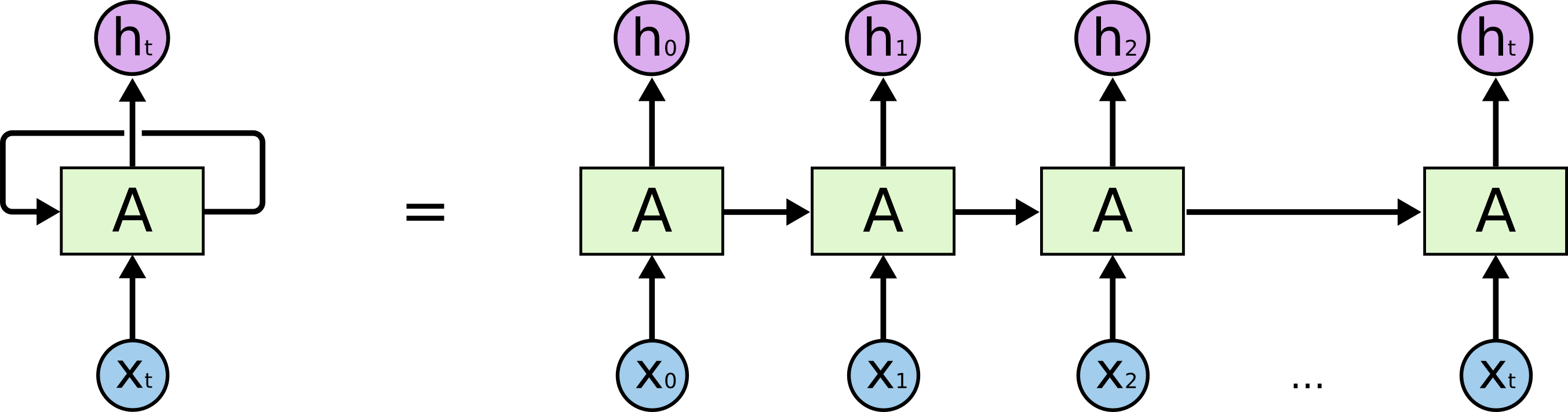上图每一次运算都是一个时间步，一共进行了 `t` 个时间步。每一个时间步输入 `xt`，得到输出 `ht` 。因为循环的存在，`ht` 的运算结果与 `x0``xt` 相关。这 `t` 个时间步称为一个完整的序列（Sequence），也可以称为训练数据集中的一个样本。举个例子，假设我们要用一句话预测某个结果，那么完整的这句话就是一个序列，每个词就是输入`xt`

将其用更公式化的方式表示就是：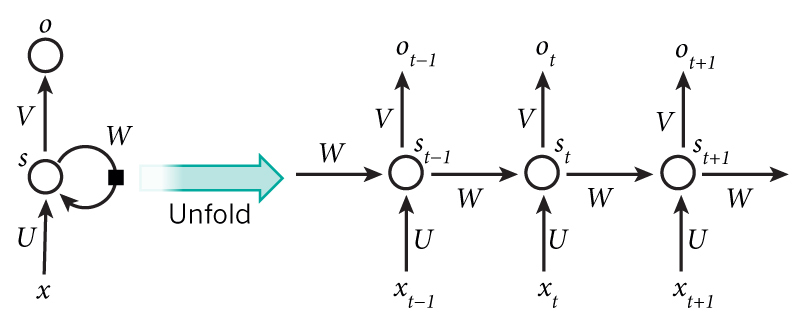1. `xt` ：时间步 `t` 的输入向量，如 `x1` 可以是代表一句话（一个序列）第二个单词的 One-Hot 向量。
2. `st`：时间步 `t` 的输入向量 RNN 的隐藏状态（hidden state），即网络的记忆。`st` 计算依赖于上一个状态 `st-1` 和当前输入：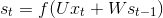f 为非线性激活函数如 `tanh``ReLU`
3. `ot`（即上上图的 `ht`）：时间步 `t` 的输出，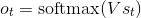这些本质上就是一些矩阵的运算，具体介绍和实现可以看这里

注意上图我们每一个时间步都会得到一个输出`ht``ot`）。但实际情况我们可能会舍弃某些输出，只取我们感兴趣的部分。大概可以分为以下几类：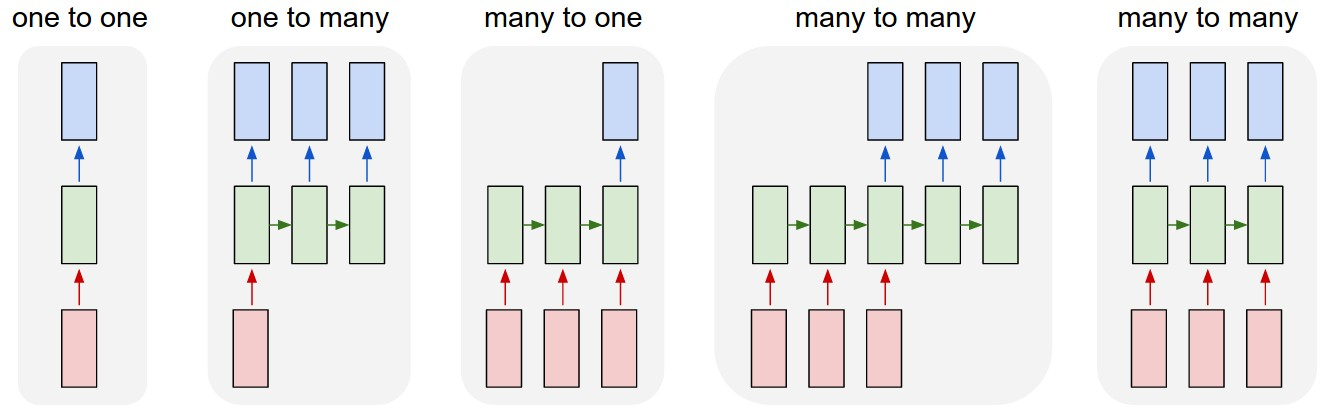1. 是没有采用 RNN 的情形。如图像分类。
2. 输入一个，输出一个序列（保留每个时间步的结果）。如输入一张图片，输出对这张图片的描述（Sequence）。
3. 输入序列，只取最后一个时间步的结果。如输入一句话，来判断这句话的情感是消极的还是积极的，显然最后一个时间步结果最可靠，因为每个单词都进行了考虑。再比如，把一张图片看成序列，对图片分类，后面的例子就是用的这种。
4. 输入序列，输出序列。如机器翻译，很好理解。
5. 同步的（Synced ）序列输入和序列输出。如给视频的每一帧标上标签。

注意，每一个时间步的长度不一定固定，理论上我们可以“无限向右扩展”。 RNN 已经取得了很多成果：翻译，语音识别，语言建模，描述图片等，但普通的 RNN 很难训练，因为存在 梯度消失梯度爆炸 等问题。而 RNN 的变体，LSTM 正是为了解决此类问题诞生的。

## LSTM

LSTM，全称为长短期记忆网络(Long Short Term Memory Networks)，是一种特殊的RNN，能够学习到长期依赖关系。LSTM由Hochreiter & Schmidhuber (1997)提出，许多研究者进行了一系列的工作对其改进并使之发扬光大。LSTM在许多问题上效果非常好，现在被广泛使用。

普通 RNN 于 LSTM 的区别在于，RNN 只有一层（如单个tanh 层）：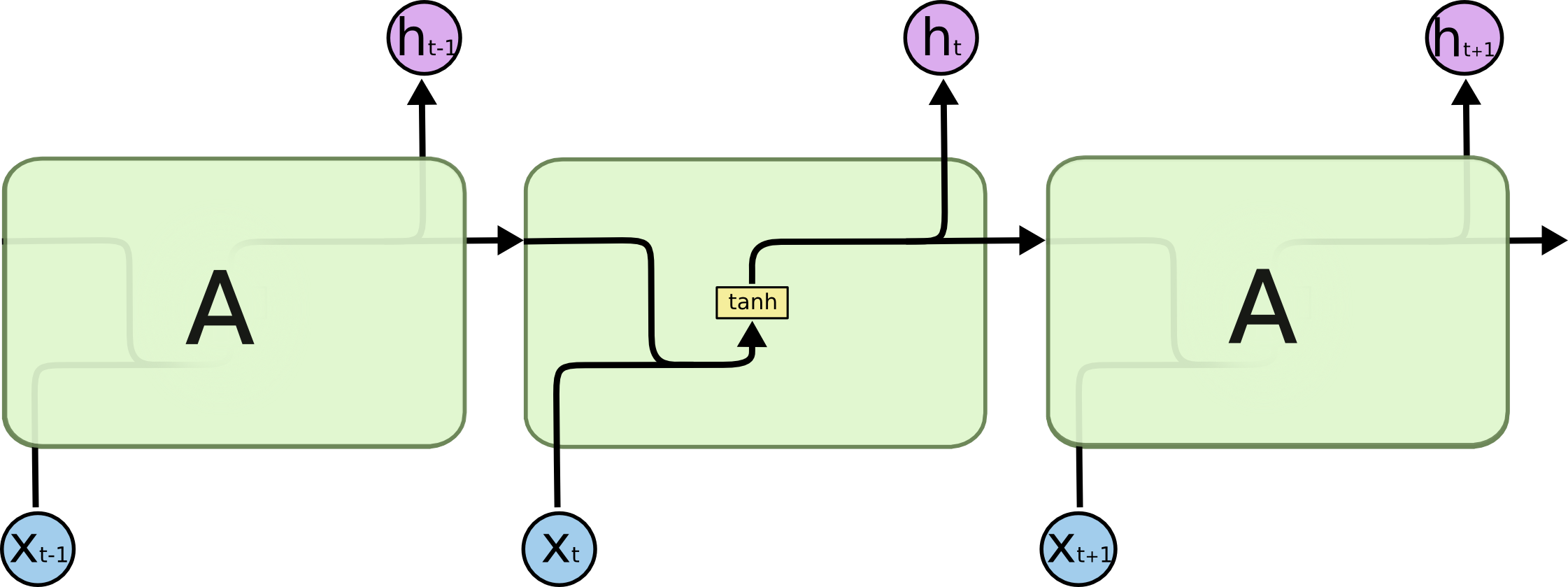LSTM 的整体结构（链式）与 RNN 一致，但 LSTM 内部有四层，并用一定的方式组织：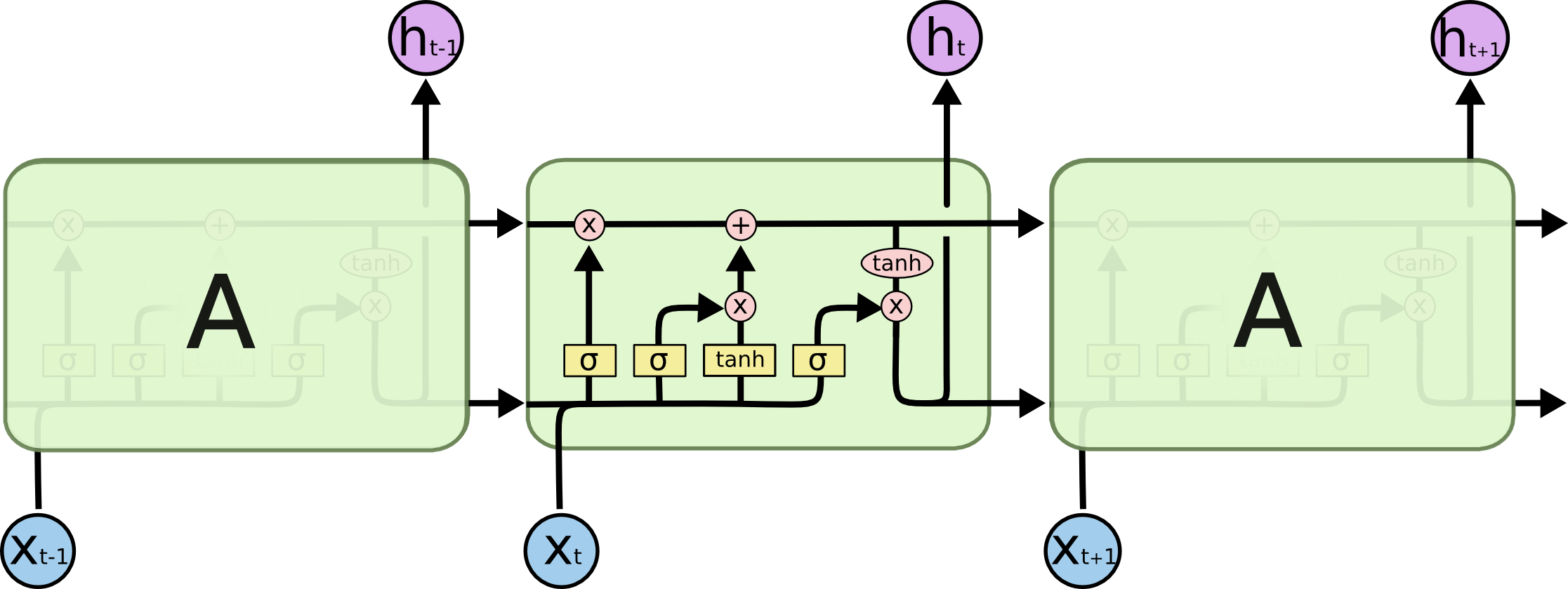关于这四层的解释，Understanding LSTM Networks 已经完美阐述了，这里只说我的理解吧。

LSTM 与 普通 RNN 的整体结构一样，都是输入 `xt`，输出 `ot`，传递给下一步 `st`区别在于 `st` 的计算。普通 RNN `st` 的计算简单，而 LSTM 为了解决长期依赖关系，引入了三个“门”：Input, forget and output gates，因此计算也变得稍微复杂：虽然六个公式看起来复杂，但是 `i``f``o`（即 input, forget and output gates）的计算公式是一样的，都是只与当前输入和上一状态有关，只是所用的参数不同。而 `g` 叫做 “候选隐藏状态”，因为 `g` 和普通 RNN 的隐藏状态 `st` 计算方法一样。为什么时候选的呢？关键在于 `ct``ct` 就是 LSTM 的“内部记忆”（Internal Memory），`ct` 计算公式很有意思： `ct-1` 乘以 `f` 加上 `g` 乘以 `i` （这里的乘法不是矩阵相乘，而是Pointwise Operation，即元素对应相乘），即忘掉 `ct-1` 中应该忘掉的，输入 `g` 中应该输入的。这就是 input gate 和 forget gate 的来历。试想 `f` 全为 0，相当于完全忘记 `ct-1``i` 全为1，相当于取 `g` 的所有作为 `ct`。因为 `f``i` 通常由 `sigmod` 函数获得，因此介于 0~1，即这两个极端之间。所以最后 `st` 的计算，就是`tanh(ct)` 乘以（Pointwise Operation）`o`，只取值得输出的。因此，这些公式没那么复杂，input, forget and output gates 就是为了对 `st` 的计算加以限制，忘掉无用的久远的记忆，过滤输入中有价值的，输出我们想要的结果 。

整个解释，这一个图完美概括了：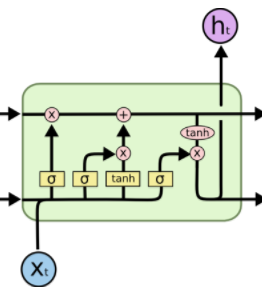所以，一定要看Understanding LSTM Networks

## 用 LSTM 实现分类任务

RNN 解决了序列的依赖问题，如果我们把图片看成一个序列能对其完成分类吗？答案是肯定的。一张图片就是一个完整的序列，我们只取最后一个时间步的输出作为分类结果即可。

以 MNIST 数据集为例，MNIST 一张图片大小为 `28 x 28`，把每一行像素看作输入 `xt`，一共有 28 行，即 28 个时间步，正好一张图片就是一个完整的序列。

``````import tensorflow as tf
from tensorflow.contrib import rnn

# Import MNIST data
from tensorflow.examples.tutorials.mnist import input_data

lr = 0.001
training_iters = 100000
batch_size = 128
display_step = 10

n_input = 28
n_step = 28
n_hidden = 128
n_classes = 10

x = tf.placeholder(tf.float32, [None, n_step, n_input])
y = tf.placeholder(tf.float32, [None, n_classes])
w = tf.Variable(tf.random_normal([n_hidden, n_classes]))
b = tf.Variable(tf.random_normal([n_classes]))

def RNN(x):
# x (batch, n_step, n_input)
# x = tf.unstack(x, n_step, 1)
cell = rnn.BasicLSTMCell(n_hidden)

# static_rnn
# outputs: (n_step, batch, n_hidden)
# state: (batch, n_hidden), state is a tuple
# outputs, state = rnn.static_rnn(cell, x, dtype=tf.float32)
# -------------------------------------------------------
# outputs: (batch, n_step, n_hidden)
outputs, states = tf.nn.dynamic_rnn(cell, x, dtype=tf.float32)

return tf.matmul(tf.transpose(outputs, [1, 0, 2])[-1], w) + b

pred = RNN(x)

cost = tf.reduce_mean(
tf.nn.softmax_cross_entropy_with_logits(logits=pred, labels=y))

correct_pred = tf.equal(tf.argmax(pred, 1), tf.argmax(y, 1))
accuracy = tf.reduce_mean(tf.cast(correct_pred, tf.float32))

tf.summary.scalar('accuracy', accuracy)
tf.summary.scalar('loss', cost)
summaries = tf.summary.merge_all()

with tf.Session() as sess:
train_writer = tf.summary.FileWriter('logs/', sess.graph)
init = tf.global_variables_initializer()
sess.run(init)
step = 1
while batch_size * step < training_iters:
batch_x, batch_y = mnist.train.next_batch(batch_size)
batch_x = batch_x.reshape(batch_size, n_step, n_input)
sess.run(optimizer, feed_dict={x: batch_x, y: batch_y})
if step % display_step == 0:
acc, loss = sess.run(
[accuracy, cost], feed_dict={x: batch_x,
y: batch_y})
print("Iter " + str(step * batch_size) + ", Minibatch Loss= " + \
"{:.6f}".format(loss) + ", Training Accuracy= " + \
"{:.5f}".format(acc))
if step % 100 == 0:
s = sess.run(summaries, feed_dict={x: batch_x, y: batch_y})

step += 1
print("Optimization Finished!")

test_len = 128
test_data = mnist.test.images[:test_len].reshape((-1, n_step, n_input))
test_label = mnist.test.labels[:test_len]
print("Testing Accuracy:", \
sess.run(accuracy, feed_dict={x: test_data, y: test_label}))
``````

训练结果：

``````.............................................................
Iter 87040, Minibatch Loss= 0.107965, Training Accuracy= 0.96875
Iter 88320, Minibatch Loss= 0.169673, Training Accuracy= 0.96094
Iter 89600, Minibatch Loss= 0.115446, Training Accuracy= 0.96875
Iter 90880, Minibatch Loss= 0.106136, Training Accuracy= 0.96094
Iter 92160, Minibatch Loss= 0.199402, Training Accuracy= 0.96094
Iter 93440, Minibatch Loss= 0.061823, Training Accuracy= 0.98438
Iter 94720, Minibatch Loss= 0.132453, Training Accuracy= 0.95312
Iter 96000, Minibatch Loss= 0.044499, Training Accuracy= 1.00000
Iter 97280, Minibatch Loss= 0.161864, Training Accuracy= 0.94531
Iter 98560, Minibatch Loss= 0.114365, Training Accuracy= 0.95312
Iter 99840, Minibatch Loss= 0.127909, Training Accuracy= 0.96094
Optimization Finished!
Testing Accuracy: 0.992188
``````

可以看到，取得了 `0.992188` 的准确率，训练时间也仅在 88 秒左右。

## 用 LSTM 实现回归任务

给定序列 `sin(x)`，求序列 `cos(x)`

``````import tensorflow as tf
from tensorflow.contrib import rnn
import numpy as np
import matplotlib.pyplot as plt

BATCH_START = 0
TIME_STEPS = 20
BATCH_SIZE = 50
INPUT_SIZE = 1
OUTPUT_SIZE = 1
CELL_SIZE = 16
LR = 0.006

def get_batch():
global BATCH_START, TIME_STEPS
x = np.arange(BATCH_START, BATCH_START + BATCH_SIZE * TIME_STEPS).reshape(
(BATCH_SIZE, TIME_STEPS)) / (10 * np.pi)

sinx = np.sin(x)
cosx = np.cos(x)

BATCH_START += TIME_STEPS
return sinx[:, :, np.newaxis], cosx[:, :, np.newaxis], x

class RNN():
def __init__(self, x):
cell = rnn.BasicLSTMCell(CELL_SIZE)
self.cell_init_state = cell.zero_state(BATCH_SIZE, dtype=tf.float32)

outputs, self.final_state = tf.nn.dynamic_rnn(
cell, x, initial_state=self.cell_init_state, time_major=False)
outputs = tf.reshape(outputs, (-1, CELL_SIZE))
w = tf.Variable(tf.random_normal([CELL_SIZE, OUTPUT_SIZE]))
b = tf.Variable(tf.random_normal([OUTPUT_SIZE]))
self.pred = tf.matmul(outputs, w) + b

def ms_error(labels, logits):
return tf.square(tf.subtract(labels, logits))

X = tf.placeholder(tf.float32, [None, TIME_STEPS, INPUT_SIZE])
Y = tf.placeholder(tf.float32, [None, TIME_STEPS, OUTPUT_SIZE])
rnn = RNN(X)
pred = rnn.pred

cost = tf.reduce_mean(
tf.square(tf.subtract(tf.reshape(pred, [-1]), tf.reshape(Y, [-1]))))
correct_pred = tf.equal(tf.argmax(pred, 1), tf.argmax(Y, 1))
accuracy = tf.reduce_mean(tf.cast(correct_pred, tf.float32))

init = tf.global_variables_initializer()
sess = tf.Session()
sess.run(init)
plt.figure(figsize=(10, 5))
plt.ion()
plt.show()
for i in range(200):
sinx, cosx, x = get_batch()
if i == 0:
feed_dict = {
X: sinx,
Y: cosx,
}
else:
feed_dict = {
X: sinx,
Y: cosx,
rnn.cell_init_state: state,
}
_, state, outputs = sess.run(
[optimizer, rnn.final_state, pred], feed_dict=feed_dict)

plt.plot(x[0, :], cosx.flatten(), 'r', x[0, :],
outputs.flatten()[:TIME_STEPS], 'b--')
plt.ylim((-1.2, 1.2))
plt.xlim(x[0, 0] - 10, x[0, -1])
plt.draw()
plt.pause(0.1)

if i % 20 == 0:
print('cost: ', sess.run(cost, feed_dict=feed_dict))

``````

可以看到一开始并不能完整的拟合：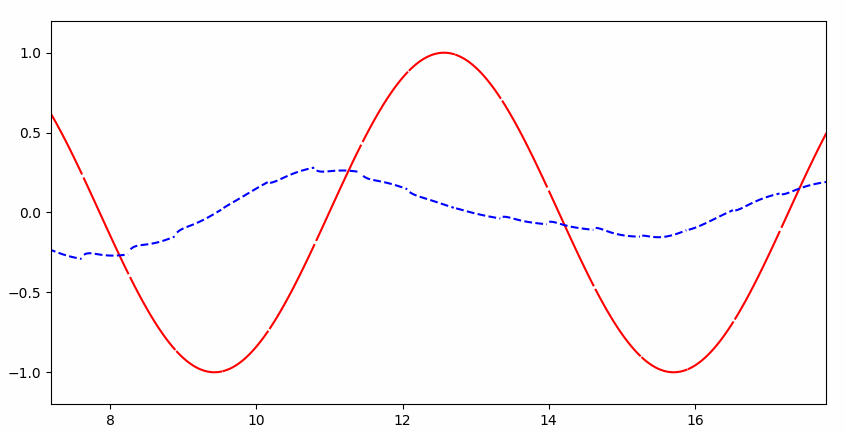但是很快就几乎完美拟合：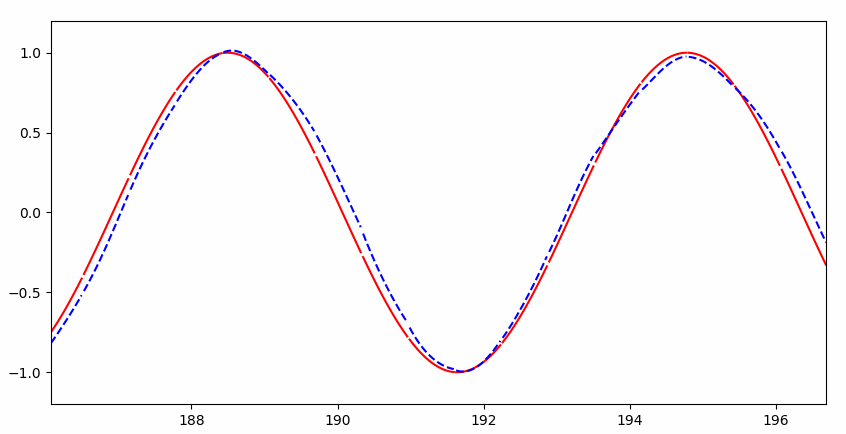## RNN 与普通神经网络的直观区别

回到上个问题，我们给定序列 `sin(x)`，求序列 `cos(x)`，实际上是已知 `cos(x)` 的值，求 `x` 的值。大家都知道，已知 `cos(x)` 的值，可能会有两个 `x` 与之对应，进而 `sin(x)` 会有两个值（下图两个小黑点）：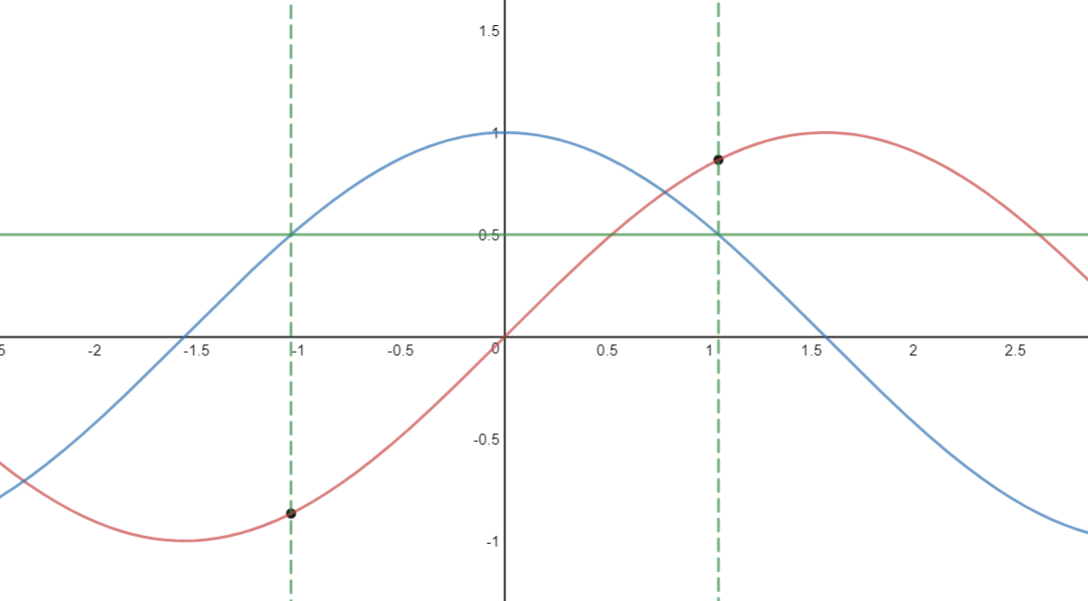这样传统的神经网络就无法解决这类函数问题了，而 RNN 之所以能解决，是因为 RNN 知道 `cos(x)` 的值的时候，会根据先前的 `cos(x)` 的值，判断在当前点上升或者下降的趋势（递增递减），进而推断出 `sin(x)` 的值。当然这只是拟人的说法，本质还是 RNN 解决了依赖关系。

如果硬让普通神经网络解决这个问题呢？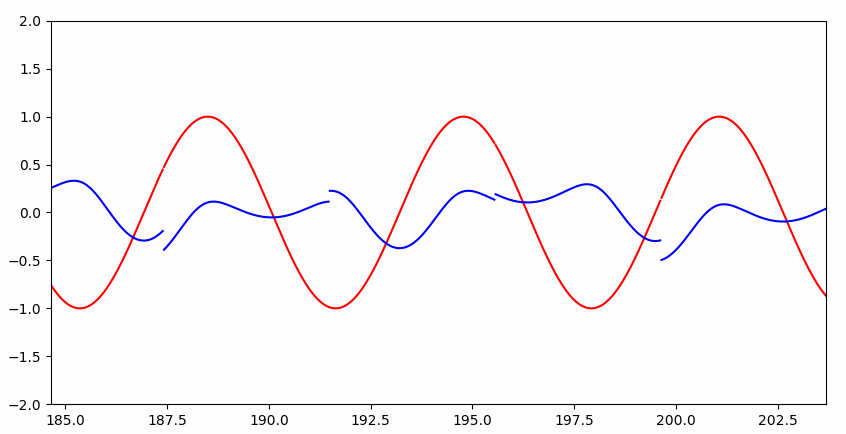Interesting~~

## 参考

凡是讲到与 RNN 有关的问题，99.9% 的文章都要引用这两篇：

所以，还不赶紧去看这两篇文章？还有一个系列：Recurrent Neural Networks Tutorial, Part 1 – Introduction to RNNs 也超级棒。

• 关于 uWSGI ，可以先看这篇文章。简单来说，WSGI 是一个 Python 协议，定义了应用程序（我们写的软件）如何与 Web 服务器（如 Nginx）通信，WSGI 只是一个接口。而 uWSGI 是一个支持多种语言的服务器容器，使用 WSGI 定义的标准实现与多种 Web 服务器的通信，并将 Web 服务器发来的请求“翻译”成应用程序所能理解形式。

### 安装

Python 2：

``````sudo apt-get update
sudo apt-get install python-pip python-dev nginx
``````

Python 3：

``````sudo apt-get update
sudo apt-get install python3-pip python3-dev nginx
``````

``````pip install uwsgi flask
``````

创建一个简单的 Flask 项目 `~/myproject/myproject.py`

``````from flask import Flask

@app.route("/")
def hello():
return "<h1 style='color:blue'>Hello There!</h1>"

if __name__ == "__main__":
app.run(host='0.0.0.0')
``````

创建启动文件为 `~/myproject/run.py`

``````from myproject import app

if __name__ == "__main__":
app.run()
``````

运行 `python run.py` ，然后访问 `http://server_domain_or_IP:5000` 将会看到：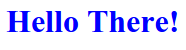当然直接使用 `python run.py` 的方法只适合本地开发，线上的话速度太慢，我们需要使用 `uwsgi`

### uwsgi

首先确保你安装了 `uwsgi`，然后运行：

``````uwsgi --socket 0.0.0.0:5000 --protocol=http -w run:app
``````

`protocol` 说明使用 `http` 协议，`-w` 指明了要启动的模块，`run` 就是项目启动文件 `run.py` 去掉扩展名，`app``run.py` 文件中的变量 `app`，即 Falsk 实例。然后访问 `http://server_domain_or_IP:5000`，同样会看到上图。说明 `uwsgi` 可以正常运行。

但是这样的话每次都从命令行启动太麻烦，可以在 `~/myproject/`目录下创建一个配置文件 `myproject.ini`

``````[uwsgi]
module = run:app
master = true
processes = 3

chdir = /home/ubuntu/myproject
socket = /path/to/sock/myproject.sock
logto = /home/to/log/ishuhui.log
chmod-socket = 660
vacuum = true
``````
• `processes = 5` 说明要启动5个子进程处理请求；`module = run:app` 和命令行使用的意义一样；
• `chdir = /home/ubuntu/myproject` 只想我们项目的根目录，即 `run.py` 所在文件夹；
• `socket = /path/to/sock/myproject.sock``uwsgi` 启动后所需要创建的文件，这个文件用来和 Nginx 通信，后面会在配置 Nginx 时用到，所以 `chmod-socket = 660` 是为了修改 `.sock` 文件权限来和 Nginx 通信；
• `logto = /home/to/log/ishuhui.log` 指明了 `uwsgi` 日志目录，`uwsgi` 会将请求历史写入该文件。

配置完成后运行：

``````uwsgi --ini myproject.ini
``````

可以看到 `/path/to/sock/myproject.sock` 目录下多了 `myproject.sock` 文件，用来和 Nginx 通信。接下来配置 Nginx。

### 配置 Nginx

配置 Nginx 特别简单，找到 Nginx 配置文件（`sudo vim /etc/nginx/sites-available/default`），修改为如下格式：

``````server {
listen 80;
server_name server_domain_or_IP;

location / {
include uwsgi_params;
uwsgi_pass unix:/path/to/sock/myproject.sock;
}
}
``````

其实重要的就两行：

``````include uwsgi_params;
uwsgi_pass unix:/path/to/sock/myproject.sock;
``````

接下来不出意外，访问 80 端口，就可以看到你的程序了。

### 将 `uwsgi` 设置为系统服务

我们运行 `uwsgi --ini myproject.ini` 之后，按 `ctrl+c` 或者关闭 ssh 连接窗口，都会导致 `uwsgi` 进程关闭。 `uwsgi` 进程一关闭，`.sock` 文件就会消失，这时访问网站 Nginx 就会报错：这时，我们需要进程管理软件管理 `uwsgi` 进程的运行了。Ubuntu 自带的 systemd 是最简单的方法，可以将我们的项目变为系统服务。首先创建 `myproject.service` 文件 `sudo vim /etc/systemd/system/myproject.service`

``````[Unit]
Description=uWSGI instance to serve myproject
After=network.target

[Service]
User=lufficc
Group=www-data
WorkingDirectory=/home/ubuntu/myproject
ExecStart=/usr/local/bin/uwsgi --ini /home/ubuntu/myprojectenv/ishuhui.ini

[Install]
WantedBy=multi-user.target
``````
• `WorkingDirectory`： 你的项目目录。
• `Environment`：需要的环境变量，比如指明你的项目的配置文件
• `ExecStart`：服务启动的代码
• `WantedBy=multi-user.target`：指明会跟随系统启动而启动该服务。

注意以上所有路径为`绝对路径`

接下来可以愉快的启动了(myproject 就是 `myproject.service` 文件名去掉扩展名)：

``````sudo systemctl start myproject
sudo systemctl restart myproject
sudo systemctl stop myproject
``````

### 参考：

2. https://www.digitalocean.com/community/tutorials/how-to-set-up-uwsgi-and-nginx-to-serve-python-apps-on-ubuntu-14-04
• 小站以前采用的是 StartSSL 的 https 证书，但是 Mozilla 封杀 StartSSL 后一直没有换。前几天终于被 Chrome 报不安全了，于是换成了 Let’s Encrypt

但是 Let’s Encrypt 证书只有90天的有效期，有没有什么便捷的方法一键生成证书呢？答案是 Certbot。Certbot 真的是便捷，不用去 Let’s Encrypt 注册账号（它会自动帮你注册），不用手动修改配置服务器配置，一行命令搞定。。。以我的服务器为例（Nginx on Ubuntu 14.04），首先安装 Certbot ：

``````\$ sudo apt-get update
\$ sudo apt-get install software-properties-common
\$ sudo apt-get update
\$ sudo apt-get install python-certbot-nginx
``````

安装好之后，只需要运行一行代码：

``````\$ sudo certbot --nginx
``````

剩下的一切会自动完成。Certbot 会自动帮你注册账户，检测 Nginx 配置文件中的域名，询问你为哪些域名生成证书，是否将 Http 重定向到 Https 等等，最后帮你自动修改 Nginx 配置并重启，这时你的网站已经变成了 Https。另外由于证书只有三个月的有限期，你还可以运行 `sudo certbot renew --dry-run`，Certbot 会帮你启动一个定时任务，在证书过期时自动更新。

还有，如果你只想生成证书怎么办？比如七牛云加速域名的证书，Nginx 配置中并没有此域名。同样一行代码搞定：`certbot certonly --cert-name example.com`。Certbot 会启动一个临时服务器来完成验证（会占用80端口或443端口，因此需要暂时关闭 Web 服务器），然后 Certbot 会把证书以文件的形式保存，包括完整的证书链文件和私钥文件。

你用的是其他操作系统和 Web 服务器怎么办？官方有详细的文档，你可以在首页选择你的软件查看对应的文档。既然这么简单就可以升级到 Https ,为什么不使用更加安全的 Https 呢？行动起来吧~

• 2013 年伦敦的一家小公司 DeepMind 发表了一篇论文 Playing Atari with Deep Reinforcement Learning 。论文描述了如何教会电脑玩 Atari 2600 游戏（仅仅让电脑观察游戏的每一帧图像和接受游戏分数的上升作为奖励信号）。结果很令人满意，因为电脑比大多数人类玩家玩的好，而且该模型在没有任何改变的情况下，学会了玩其他游戏，并且在三个游戏中表现比人类玩家好！自此通用人工智能的话题开始火热 – 能够适应各种负责环境而不仅仅局限于玩棋类游戏，而 DeepMind 因此被谷歌看中而被收购。2015 年，DeepMind 又发表了一篇 Human-level control through deep reinforcement learning，在本篇论文中 DeepMind 用同样的模型，教会电脑玩49种游戏，而且过半游戏比专业玩家玩得更好。2016年3月，AlphaGo 与围棋世界冠军、职业九段选手李世石进行人机大战，并以4:1的总比分获胜；2016年末2017年初，该程序在中国棋类网站上以“大师”（Master）为注册帐号与中日韩数十位围棋高手进行快棋对决，连续60局无一败绩。

自此，在机器学习领域，除了监督学习和非监督学习，强化学习（Reinforcement Learning）也逐渐走进人们的视野。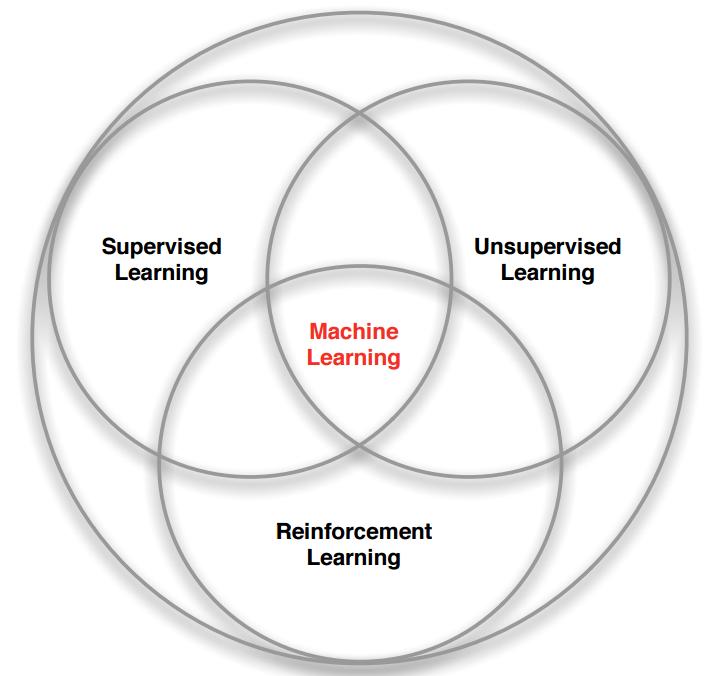{.to-figure}

## 强化学习

以打砖块游戏为例，游戏中你控制底部的挡板来反弹小球，来清除屏幕上半部分的砖块。每次你打中砖块，分数增加，你也得到一个奖励，而没有接到小球则会受到惩罚。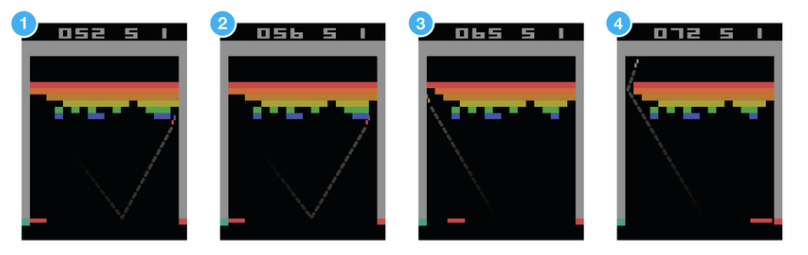{.to-figure}

假设让一个神经网络来玩这个游戏， 输入是屏幕图像，输出将是三个动作：左，右或发射球。很明显这是一个分类问题，对于每一帧图像，我们计算到到一个动作即可（为屏幕数据分类）。 但是听起来很简单，实际上有很多有挑战性的细节。因为我们当前的动作奖励有可能是在此之后一段时间获得的。不像监督学习，对于每一个样本，都有一个确定的标签与之对应，而强化学习没有标签，只有一个时间延迟的奖励，而且游戏中我们往往牺牲当前的奖励来获取将来更大的奖励。因为我们的小球在打到砖块，获得奖励时，事实上挡板并没有移动，该奖励是由于之前的一系列动作来获得的。这就是信用分配问题（Credit Assignment Problem），即当前的动作要为将来获得更多的奖励负责。

而且在我们找到一个策略，让游戏获得不错的奖励时，我们是选择继续坚持当前的策略，还是探索新的策略以求更多的奖励？这就是探索与开发（Explore-exploit Dilemma）的问题。

强化学习就是一个重要的解决此类问题的模型，它是从我们的人类经验中总结出来的。在现实生活中，如果我们做某件事获得奖励，那么我们会更加偏向于做这件事。比如你的狗狗早上给你把鞋子叼过来，你对他说 Good dog 并奖励它，那么狗狗会更加偏向于叼鞋。而且人类每天在学校的成绩、在家来自父母的夸奖、还是工作上的薪水本质上都是在奖励。

## 马尔可夫决策过程 （Markov Decision Process）

那么如何用数学的方法来解决此类问题呢？最常用的方法就是那此类问题看作一个马尔可夫决策过程 （Markov Decision Process）。

MDP 中有两个对象：AgentEnvironment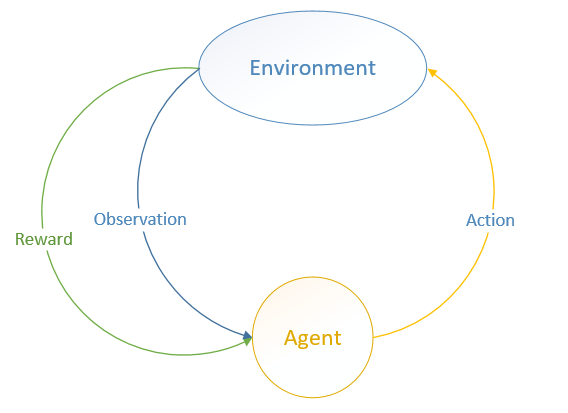Environment 处于一个特定的状态State）（如打砖块游戏中挡板的位置、各个砖块的状态等），Agent 可以通过执行特定的动作Actions ）（如向左向右移动挡板）来改变 Environment状态Environment 状态改变之后会返回一个观察Observation）给Agent，同时还会得到一个奖励Reward）（可以为负，就是惩罚），这样 Agent 根据返回的信息采取新的动作，如此反复下去。Agent 如何选择动作叫做策略Policy）。MDP 的任务就是找到一个策略，来最大化奖励。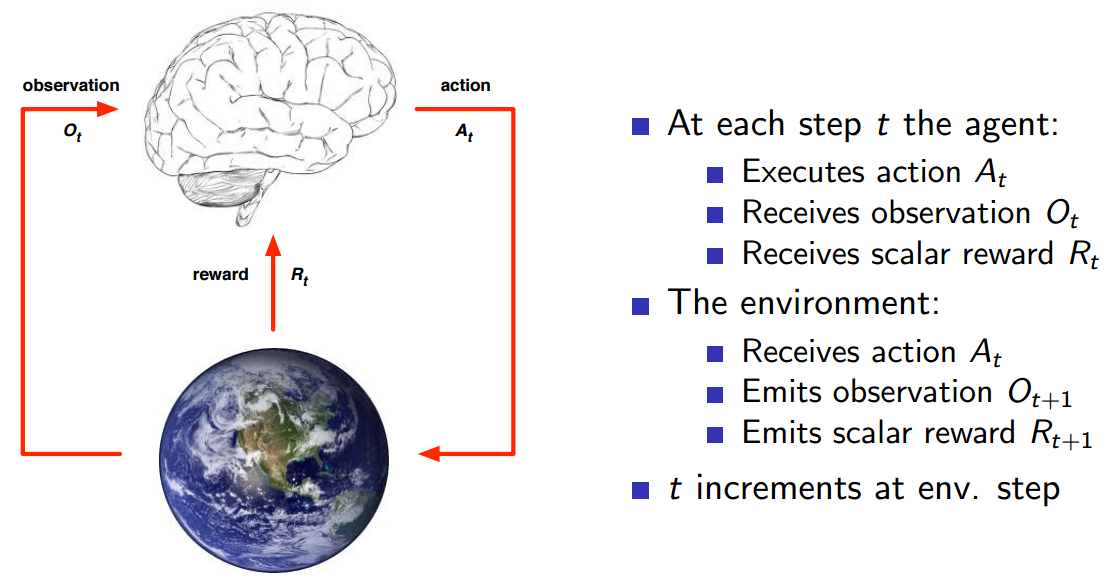具体的执行步骤如上图图所示。注意 StateObservation 区别：StateEnvironment 的私有表达，我们往往不知道不会直接到的。在 MDP 中，当前状态State （Markov state）包含了所有历史信息，即将来只和现在有关，与过去无关，因为现在状态包含了所有历史信息。举个例子，在一个遵循牛顿第二定律的世界里，我们随意抛出一个小球，某一时刻 t 知道了小球的速度和加速度，那么 t 之后的小球的位置都可以由当前状态，根据牛顿第二定律计算出来。再举一个夸张的例子，如果宇宙大爆炸时奇点的状态已知，那么以后的所有状态就已经确定，包括人类进化、我写这篇文章和你在阅读这篇文章都是可以根据那一状态推断出来的。当然这只是理想状况，现实往往不会那么简单（因为这只是马尔科夫的一个假设）。只有满足这样条件的状态才叫做马尔科夫状态。即：正是因为 State 太过于复杂，我们往往可以需要一个对 Environment 的观察来间接获得信息，因此就有了 Observation。不过 Observation 是可以等于 State 的，在游戏中，一帧游戏画面完全可以代表当前状态，因此 Observation = State，此时叫做 Full Observability。

状态、动作、状态转移概率组成了 MDP，一个 MDP 周期（episode ）由一个有限的状态、动作、奖励队列组成：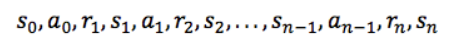这里 si 代表状态，ai 代表行动，ri + 1（下标 i+1）是执行动作后的奖励。 最终状态为sn（例如“游戏结束”）。

## 折扣未来奖励（Discounted Future Reward）

为了获得更多的奖励，我们往往不能只看当前奖励，更要看将来的奖励。

给定一个 MDP 周期，总的奖励显然为：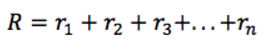那么，从当前时间 t 开始，总的将来的奖励为：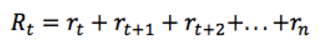但是 Environment 往往是随机的，执行特定的动作不一定得到特定的状态，因此将来的奖励所占的权重要依次递减，因此使用 discounted future reward 代替：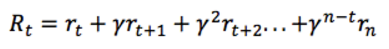这里 γ 是0和1之间的折扣因子 —— 越是未来的奖励，折扣越多，权重越小。而明显上式是个迭代过程，因此可以写作：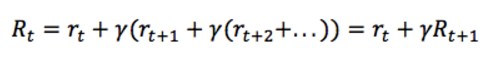即当前时刻的奖励等于当前时刻的即时奖励加上下一时刻的奖励乘上折扣因子 γ。如果 γ 等于0，意味着只看当前奖励；如果 γ 等于1，意味着环境是确定的，相同的动作总会获得相同的奖励。因此实际中 γ 往往取类似0.9这样的值。因此我们的任务变成了找到一个策略，最大化将来的奖励 R

## Q-learning

在 Q-learning 中，我们定义一个函数 Q(s，a)，表示在状态 s 执行动作 a 时的最大折扣未来奖励，并从此刻开始优化它：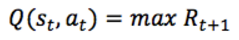Q(s，a) 看作是在状态 s，执行动作 a时，游戏结束时的分数就行了。不要惊奇为什么在状态 s 就可以知道游戏结束时的分数，因为这是马尔科夫过程，将来只和现在有关，现在是将来的充分条件。这样最大化 Q(s，a) 就相当于最大化我们游戏的得分了（至于为什么叫做 Q，因为它代表了在特定状态 s 下特定动作 a 的质量”quality”）。

假设你处于状态 s，思考应该采取行动 ab 以在比赛结束时获得最高分的动作。一旦有了 Q函数，答案就变得一目了然 —— 选择 Q 值最高的动作：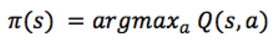这里的 π 代表策略，代表我们如何在某状态选取动作。

怎么才能得到这个Q函数呢？ 只关注一个转换 <s，a，r，s’>， 就像上一节中折扣未来奖励一样，我们可以用下一个状态的Q值表示：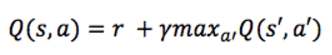这就是贝尔曼方程（Bellman equation），当前状态 s 的最大将来奖励等于下一状态 s’ 的最大将来奖励乘以折扣因子。这样我们就可以用贝尔曼方程来近似了，最简单的方法就是把Q函数看作二维数组，行代表状态，列代表动作，那么算法描述如下：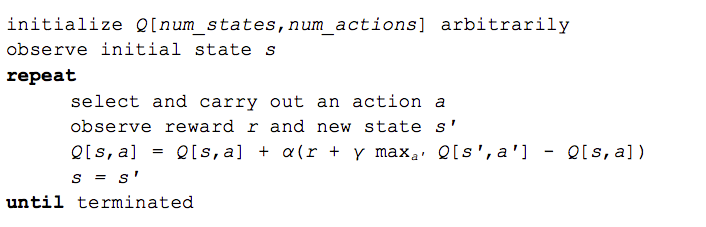{.to-figure}

α是一个学习速率（learning rate），它控制了先前的Q值和新的Q值之间的差异有多少被考虑在内。 特别地，当α = 1时，则两个 Q [s，a] 抵消，更新与贝尔曼方程完全相同。

我们用来更新 Q [s，a]maxQ [s’，a’] 一开始只是随机的，但随着迭代，会慢慢收敛，最终近似于真实值。具体的例子可以参看这里：A Painless Q-Learning Tutorial （中文版：一个 Q-learning 算法的简明教程），详细地一步一步介绍了是怎么收敛的。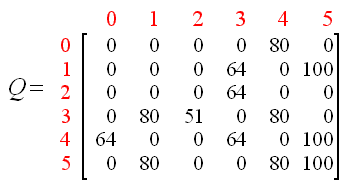{.to-figure}

## Deep Q Network

打砖块游戏中的环境状态可以由挡板的位置，球的位置和方向以及每个砖块的存在或不存在来定义。然而，这种直观的表示只能代表具体的游戏。有通用的方法适合所有的游戏吗？答案是屏幕像素 —— 它们隐含地包含关于游戏情况的所有相关信息，球的速度和方向也可以包含在连续两个屏幕像素中。

如果我们使用 DeepMind 论文中的预处理方法，将游戏画面放缩为 84×84，并将其转换为256灰度级的灰度级，我们将拥有 25684x84x4≈10的67970次方 个可能的游戏状态。这意味着Q表中有10的67970次方 行 —— 超过已知宇宙中的原子数！即使某些像素组合永远不会发生 —— 我们可以将其表示为仅包含访问状态的稀疏矩阵，但是，状态还是很多，而且难以收敛。

这时候深度学习登场了，神经网络可以高效的表示高维数据，将其映射为低维数据。 因此我们可以用神经网络代表我们的Q函数，将状态（游戏画面）和动作作为输入，并输出相应的Q值。 或者，我们只能将游戏画面作为输入，并输出每个可能动作的Q值。 这种方法的优点是，如果我们要执行Q值更新或选择具有最高Q值的动作，我们只需要通过网络进行一次前进传播，并且可以立即获得所有动作的Q值 。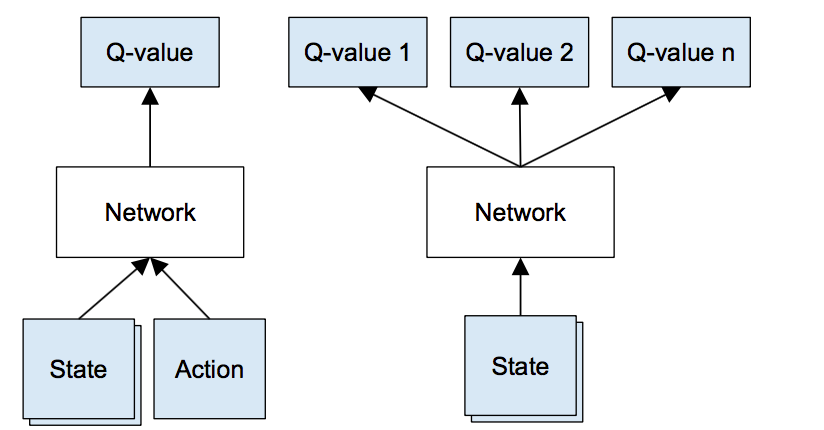左：Q函数的公式化表示；右：DeepMind 使用的方便用神经网络表示的结构，输出的Q值与动作一一对应

输入是四个 84×84 灰度级游戏画面，输出是每个可能动作的Q值（在Atari中为18）。 Q值可以是任何实际值，这使得它成为一个回归任务，可以用简单的平方误差损失进行优化：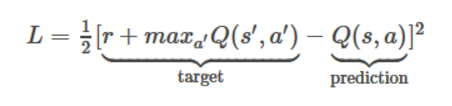给定转换 <s，a，r，s’>，Q表算法需要修改为：

1. 对当前状态 s 进行前向传播以获取所有动作的Q值。
2. 对下一个状态 s’ 进行前向传播，并计算最大Q值 max Q(s’, a’)
3. 将动作对应的目标Q值设置为 r + γ max Q（s’，a’）（步骤2中计算的最大值）。
4. 使用反向传播算法更新参数。

## 经验回放 （Experience Replay）

现在我们使用卷积神经网络近似Q函数。但事实证明，使用非线性函数近似Q值不是非常稳定，而且难以收敛，在单GPU上需要很长时间，差不多一个星期才会看到成效。

有很多技巧可以优化，最重要的技巧是经验回放。在游戏过程中，所有的经历 <s，a，r，s’> 存储起来。训练网络时，使用来自存储的经验，这打破了后续训练样本的相似性，而且可以平滑训练结果。

## 探索与开发（Exploration-Exploitation）

首先，当Q表或Q网络被随机初始化时，其预测最初也是随机的。如果我们选择具有最高Q值的动作，则该动作也将是随机的，这时 Agent 执行的动作是随机的。随着Q函数的收敛，返回更一致的Q值，探索量将减少，此时这些一致的Q值叫做开发（Exploitation）。但是这些探索是“贪心”的，它发现第一个有效策略后停止收敛，很有可能我们只是找到了一个局部最优解。

对于上述问题，一个简单而有效的解决方案是 ε-greedy exploration *—— 以概率 *ε 选择一个随机动作，否则选择具有最高Q值“贪婪”动作。DeepMind 实际上将 ε 随时间从1减少到0.1，一 开始，系统完全随机移动，最大限度地探索状态空间，然后稳定的利用开发率。

最终带有经验回放的算法如下：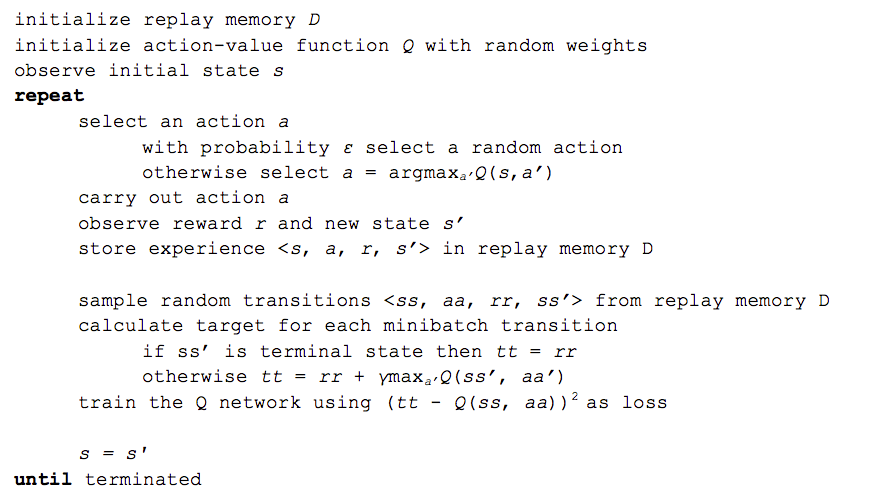DeepMind 还有更多的技巧来优化，如目标网络，错误剪辑，奖励剪辑等，但这些都不在此介绍范围之内。

## 实现

下面使用 TensorFlow 实现 Q 算法，解决 OpenAI GymCartPole 游戏和 FlappyBird 游戏。

Q 算法的 Q函数我么可以用任何函数来代替，不限于神经网络或者卷积神经网络，因此我们需要把它抽象出来：

``````class Model():
def __init__(self, num_outputs):
self.num_outputs = num_outputs

def definition(self):
raise NotImplementedError

def get_num_outputs(self):
return self.num_outputs
``````

`Model` 代表了Q函数的抽象，`num_outputs` 代表了可能的动作个数，CartPole 中有两个：左移或者右移；FlappyBird 也有两个动作：点击屏幕或者什么都不做。`definition` 函数代表具体的Q函数定义，返回输入和输出。

因为游戏是不确定的，所以我们需要抽象一个 `Env` :

``````# Env-related abstractions
class Env():
def step(self, action_index):
raise NotImplementedError

def reset(self):
raise NotImplementedError

def render(self):
raise NotImplementedError
``````

`Env` 可以是任何游戏，或者其他问题。`step` 函数表示执行指定动作 `action_index`，然后返回一个元组：`(state, reward, terminal, info)``state` 是游戏当前状态，如屏幕像素；`reward` 是奖励，可以为负数； `terminal` 代表游戏是否结束；`info` 代表一些其他信息，可有可无。

然后是我们的算法实现：

``````import numpy as np
import tensorflow as tf
import random
import numpy as np
import time
import sys
import collections

class DeepQNetwork():
def __init__(self,
model,
env,
learning_rate=0.001,
gamma=0.9,
replay_memeory_size=10000,
batch_size=32,
initial_epsilon=0.5,
final_epsilon=0.01,
decay_factor=1,
logdir=None,
save_per_step=1000,
test_per_epoch=100):
self.model = model
self.env = env
self.num_actions = model.get_num_outputs()
self.learning_rate = learning_rate
self.optimizer = optimizer
self.gamma = gamma
self.epsilon = initial_epsilon
self.initial_epsilon = initial_epsilon
self.final_epsilon = final_epsilon
self.decay_factor = decay_factor
self.logdir = logdir
self.test_per_epoch = test_per_epoch

self.replay_memeory = collections.deque()
self.replay_memeory_size = replay_memeory_size
self.batch_size = batch_size
self.define_q_network()
# session
self.sess = tf.InteractiveSession()
self.sess.run(tf.global_variables_initializer())
if self.logdir is not None:
if not self.logdir.endswith('/'): self.logdir += '/'
self.save_per_step = save_per_step
self.saver = tf.train.Saver()
checkpoint_state = tf.train.get_checkpoint_state(self.logdir)
if checkpoint_state and checkpoint_state.model_checkpoint_path:
path = checkpoint_state.model_checkpoint_path
self.saver.restore(self.sess, path)
print('Restore from {} successfully.'.format(path))
else:
print('No checkpoint.')
self.summaries = tf.summary.merge_all()
self.summary_writer = tf.summary.FileWriter(
self.logdir, self.sess.graph)
sys.stdout.flush()

def define_q_network(self):
self.input_states, self.q_values = self.model.definition()
self.input_actions = tf.placeholder(tf.float32,
[None, self.num_actions])
# placeholder of target q values
self.input_q_values = tf.placeholder(tf.float32, [None])
# only use selected q values
action_q_values = tf.reduce_sum(
tf.multiply(self.q_values, self.input_actions),
reduction_indices=1)

self.global_step = tf.Variable(0, trainable=False)
# define cost
self.cost = tf.reduce_mean(
tf.square(self.input_q_values - action_q_values))
self.optimizer = self.optimizer(self.learning_rate).minimize(
self.cost, global_step=self.global_step)
tf.summary.scalar('cost', self.cost)
tf.summary.scalar('reward', tf.reduce_mean(action_q_values))

def egreedy_action(self, state):
if random.random() <= self.epsilon:
action_index = random.randint(0, self.num_actions - 1)
else:
action_index = self.action(state)
if self.epsilon > self.final_epsilon:
self.epsilon *= self.decay_factor
return action_index

def action(self, state):
q_values = self.q_values.eval(feed_dict={self.input_states:
[state]})
return np.argmax(q_values)

def do_train(self, epoch):
# randomly select a batch
mini_batches = random.sample(self.replay_memeory, self.batch_size)
state_batch = [batch for batch in mini_batches]
action_batch = [batch for batch in mini_batches]
reward_batch = [batch for batch in mini_batches]
next_state_batch = [batch for batch in mini_batches]

# target q values
target_q_values = self.q_values.eval(
feed_dict={self.input_states: next_state_batch})
input_q_values = []
for i in range(len(mini_batches)):
terminal = mini_batches[i]
if terminal:
input_q_values.append(reward_batch[i])
else:
# Discounted Future Reward
input_q_values.append(reward_batch[i] +
self.gamma * np.max(target_q_values[i]))
feed_dict = {
self.input_actions: action_batch,
self.input_states: state_batch,
self.input_q_values: input_q_values
}
self.optimizer.run(feed_dict=feed_dict)
step = self.global_step.eval()
if self.saver is not None and epoch > 0 and step % self.save_per_step == 0:
summary = self.sess.run(self.summaries, feed_dict=feed_dict)
self.summary_writer.flush()
self.saver.save(self.sess, self.logdir + 'dqn', self.global_step)

# num_epoches: train epoches
def train(self, num_epoches):
for epoch in range(num_epoches):
epoch_rewards = 0
state = self.env.reset()
# 9999999999: max step per epoch
for step in range(9999999999):
# ε-greedy exploration
action_index = self.egreedy_action(state)
next_state, reward, terminal, info = self.env.step(
action_index)
# one-hot action
one_hot_action = np.zeros([self.num_actions])
one_hot_action[action_index] = 1
# store trans in replay_memeory
self.replay_memeory.append((state, one_hot_action, reward,
next_state, terminal))
# remove element if exceeds max size
if len(self.replay_memeory) > self.replay_memeory_size:
self.replay_memeory.popleft()

# now train the model
if len(self.replay_memeory) > self.batch_size:
self.do_train(epoch)

# state change to next state
state = next_state
epoch_rewards += reward
if terminal:
# Game over. One epoch ended.
break
# print("Epoch {} reward: {}, epsilon: {}".format(
#     epoch, epoch_rewards, self.epsilon))
# sys.stdout.flush()

#evaluate model
if epoch > 0 and epoch % self.test_per_epoch == 0:
self.test(epoch, max_step_per_test=99999999)

def test(self, epoch, num_testes=10, max_step_per_test=300):
total_rewards = 0
print('Testing...')
sys.stdout.flush()
for _ in range(num_testes):
state = self.env.reset()
for step in range(max_step_per_test):
# self.env.render()
action = self.action(state)
state, reward, terminal, info = self.env.step(action)
total_rewards += reward
if terminal:
break
average_reward = total_rewards / num_testes
print("epoch {:5} average_reward: {}".format(epoch, average_reward))
sys.stdout.flush()
``````

解释一下这个算法： `__init__` 方法除了 `env``model` 是必须的，其他都是可选的。

1. `optimizer` 指定了 Tensorflow 使用的优化器；
2. `learning_rate` 是学习速率；
3. `gamma` 就是算法中的折扣因子 γ
4. `replay_memeory_size` 是存放经验的最大数量；
5. `batch_size` 是从经验池取经验用于训练的大小；
6. `epsilon` 就是 ε-greedy exploration 中的 ε，表示 `epsilon` 将从 `initial_epsilon``decay_factor` 的速率下降到 `final_epsilon` 为止，`decay_factor` 等于1表示不下降；
7. `logdir` 是 Tensorflow 保存模型的路径，为 `None` 表示不保存训练结果；
8. `save_per_step` 是每多少步保存一下断点；
9. `test_per_epoch` 是每多少不测试评估一下训练进度。

定义q network 的损失函数：

``````def define_q_network(self):
self.input_states, self.q_values = self.model.definition()
self.input_actions = tf.placeholder(tf.float32,
[None, self.num_actions])
# placeholder of target q values
self.input_q_values = tf.placeholder(tf.float32, [None])
# only use selected q values
action_q_values = tf.reduce_sum(
tf.multiply(self.q_values, self.input_actions),
reduction_indices=1)

self.global_step = tf.Variable(0, trainable=False)
# define cost
self.cost = tf.reduce_mean(
tf.square(self.input_q_values - action_q_values))
self.optimizer = self.optimizer(self.learning_rate).minimize(
self.cost, global_step=self.global_step)
tf.summary.scalar('cost', self.cost)
tf.summary.scalar('reward', tf.reduce_mean(action_q_values))
``````

然后是 `train` 方法：

``````# num_epoches: train epoches
def train(self, num_epoches):
for epoch in range(num_epoches):
epoch_rewards = 0
state = self.env.reset()
# 9999999999: max step per epoch
for step in range(9999999999):
# ε-greedy exploration
action_index = self.egreedy_action(state)
next_state, reward, terminal, info = self.env.step(
action_index)
# one-hot action
one_hot_action = np.zeros([self.num_actions])
one_hot_action[action_index] = 1
# store trans in replay_memeory
self.replay_memeory.append((state, one_hot_action, reward,
next_state, terminal))
# remove element if exceeds max size
if len(self.replay_memeory) > self.replay_memeory_size:
self.replay_memeory.popleft()

# now train the model
if len(self.replay_memeory) > self.batch_size:
self.do_train(epoch)

# state change to next state
state = next_state
epoch_rewards += reward
if terminal:
# Game over. One epoch ended.
break
# print("Epoch {} reward: {}, epsilon: {}".format(
#     epoch, epoch_rewards, self.epsilon))
# sys.stdout.flush()

#evaluate model
if epoch > 0 and epoch % self.test_per_epoch == 0:
self.test(epoch, max_step_per_test=99999999)
``````

这里我们把 `(state, one_hot_action, reward, next_state, terminal)` 元组存在队列中，如果队列长度大于 `batch_size` 就开始训练。

然后是具体的训练：

``````def do_train(self, epoch):
# randomly select a batch
mini_batches = random.sample(self.replay_memeory, self.batch_size)
state_batch = [batch for batch in mini_batches]
action_batch = [batch for batch in mini_batches]
reward_batch = [batch for batch in mini_batches]
next_state_batch = [batch for batch in mini_batches]

# target q values
target_q_values = self.q_values.eval(
feed_dict={self.input_states: next_state_batch})
input_q_values = []
for i in range(len(mini_batches)):
terminal = mini_batches[i]
if terminal:
input_q_values.append(reward_batch[i])
else:
# Discounted Future Reward
input_q_values.append(reward_batch[i] +
self.gamma * np.max(target_q_values[i]))
feed_dict = {
self.input_actions: action_batch,
self.input_states: state_batch,
self.input_q_values: input_q_values
}
self.optimizer.run(feed_dict=feed_dict)
step = self.global_step.eval()
if self.saver is not None and epoch > 0 and step % self.save_per_step == 0:
summary = self.sess.run(self.summaries, feed_dict=feed_dict)
self.summary_writer.flush()
self.saver.save(self.sess, self.logdir + 'dqn', self.global_step)
``````

首先随机选择一批数据，然后计算 Target Q 值，最后训练即可。

接下来用真实的游戏测试一下吧！

### CartPole

CartPole 比较简单，状态只是一个一个4维的实数值向量，像这样：

``````[-0.00042486 -0.02710707  0.01032103  -0.04882064]
``````

我们完全不用管这四个实数具体什么意思，管它是什么角度、加速度等，让神经网络自己理解去吧！

定义我们的 CartPoleEnv，使用 Open AI 的 Gym：

``````class CartPoleEnv(Env):
def __init__(self):
self.env = gym.make('CartPole-v0')

def step(self, action_index):
s, r, t, i = self.env.step(action_index)
return s, r, t, i

def reset(self):
return self.env.reset()

def render(self):
self.env.render()
``````

定义 Q 函数，一个简单的神经网络（4-16-2，4代表输入，16代表隐层神经元个数，2代表两个动作对应的Q值），因为 CartPole 太简单了：

``````model = SimpleNeuralNetwork([4, 16, 2])
``````

然后开始训练：

``````model = SimpleNeuralNetwork([4, 16, 2])
env = CartPoleEnv()
qnetwork = DeepQNetwork(
model=model, env=env, learning_rate=0.0001, logdir='./tmp/CartPole/')
qnetwork.train(4000)
``````

一开始完全是随机的，但随着迭代次数增加，效果越来越好（可以直接运行查看效果），而且收敛的非常快，80.0k 之后几乎完全收敛：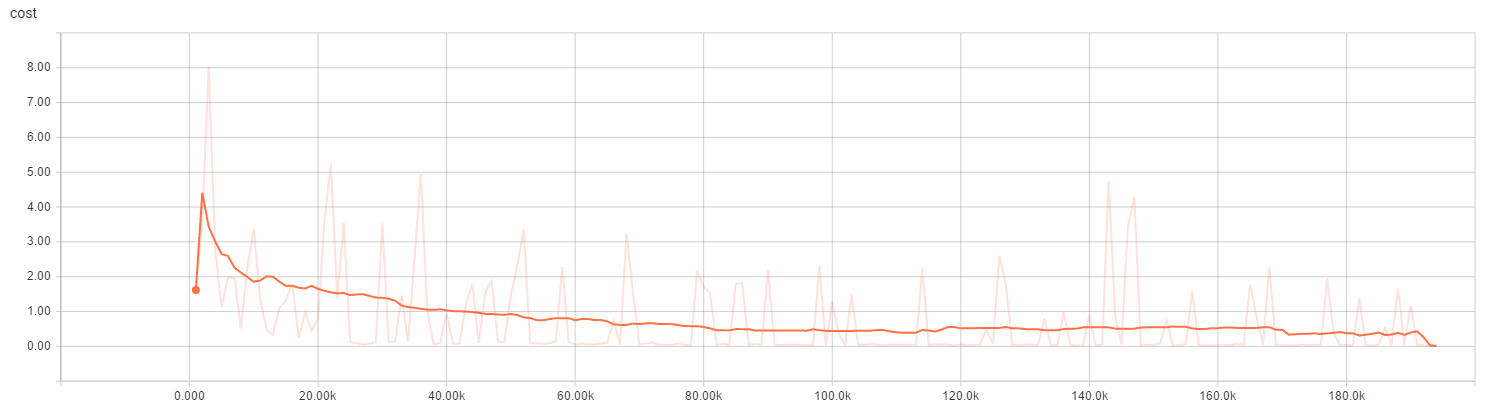CartPole损失变化曲线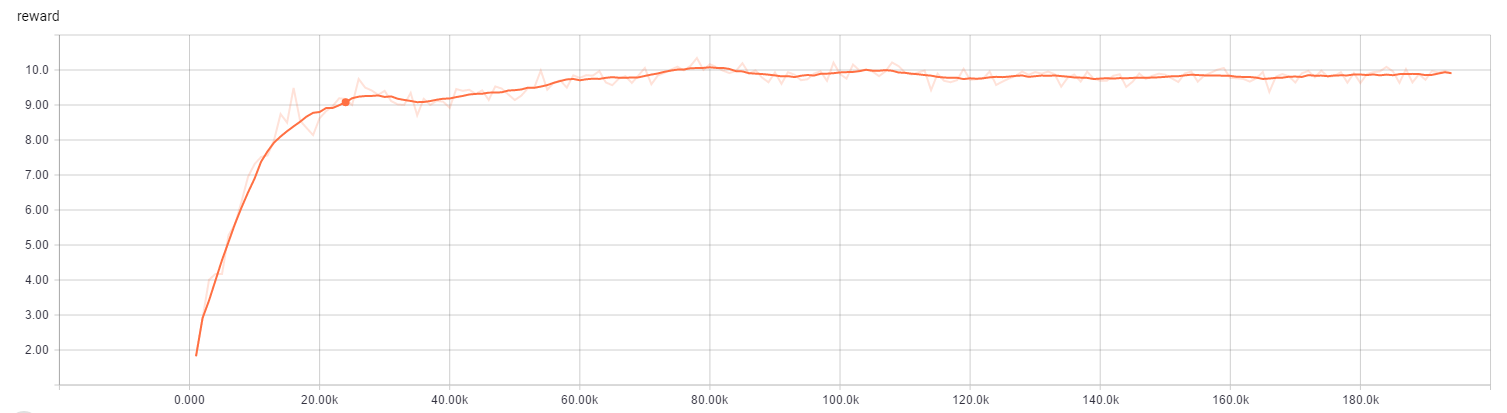CartPole奖励变化曲线

### FlappyBird

FlappyBird 比较复杂，我们采用原始屏幕像素作为输入。但是为了加快收敛，使用了一下技巧：

抽取游戏背景：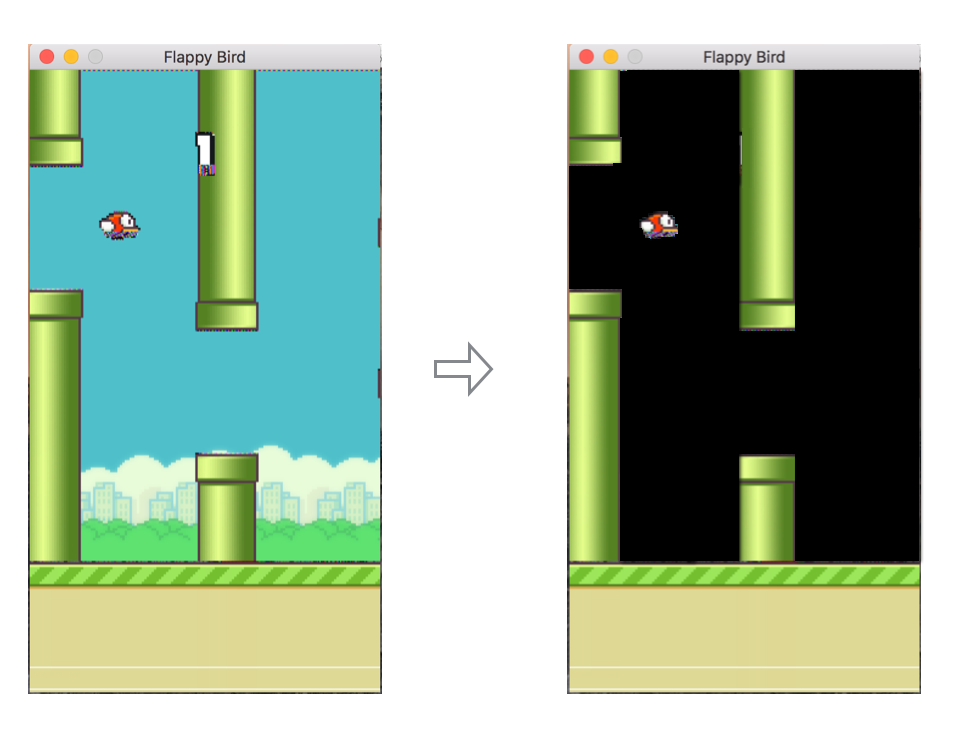裁剪掉下部无用数据：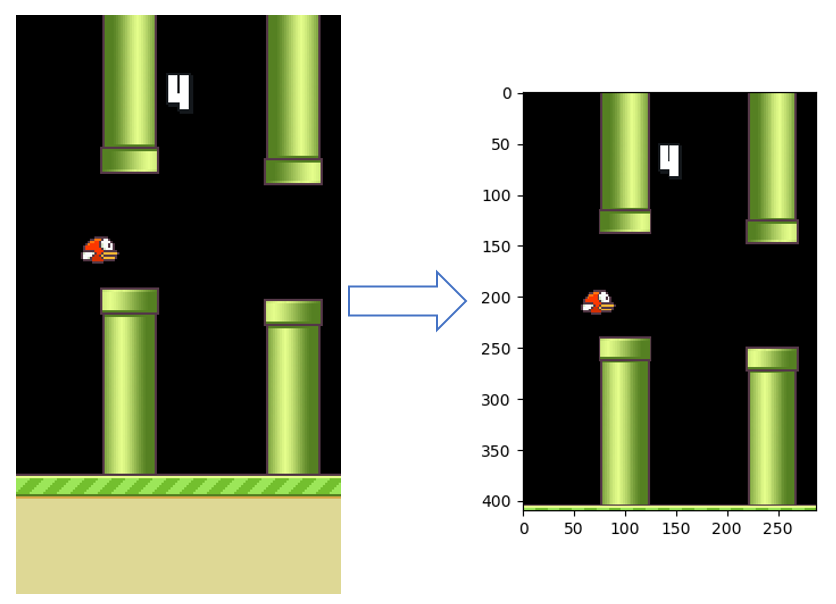放缩为64x64并二值化：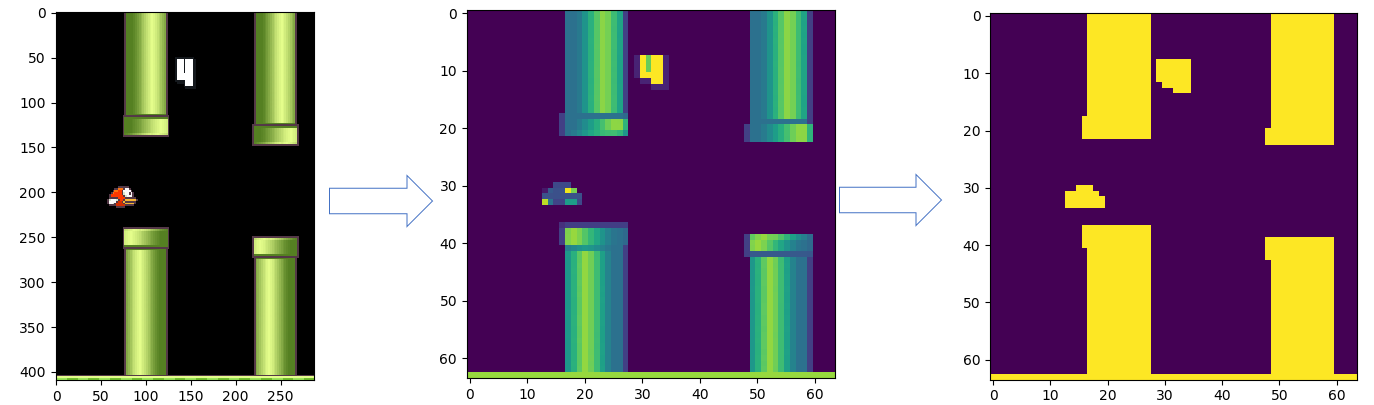然后就可以使用神经网络训练了：

``````model = CNN(img_w=image_size, img_h=image_size, num_outputs=2)
env = FlappyBirdEnv()
qnetwork = DeepQNetwork(
model=model,
env=env,
learning_rate=1e-8,
initial_epsilon=0.1,
final_epsilon=0,
decay_factor=0.99,
save_per_step=1000,
logdir='./tmp/FlappyBird/')
if train:
qnetwork.train(10000)
else:
runGame(env, network)
``````

为了加快收敛，我使用了这样一个技巧：

因为 FlappyBird 大部分时间是什么都不做的，只有在很少概率情况下才会点击屏幕，所以，在探索阶段，让它更所的几率（95%）选择什么都不做。（你不用修改也没关系，但会增加训练时间）

``````def egreedy_action(self, state):
#Exploration
if random.random() <= self.epsilon:
if random.random() < 0.95:
action_index = 0
else:
action_index = 1
# action_index = random.randint(0, self.num_actions - 1)
else:
#Exploitation
action_index = self.action(state)
if self.epsilon > self.final_epsilon:
self.epsilon *= self.decay_factor
return action_index
``````

而且训练过程中，我也动态了调整了 learning rate，让它更快的收敛。经过这些优化，很快就进行了收敛（两个小时，使用CPU训练，而不用等上一整天）：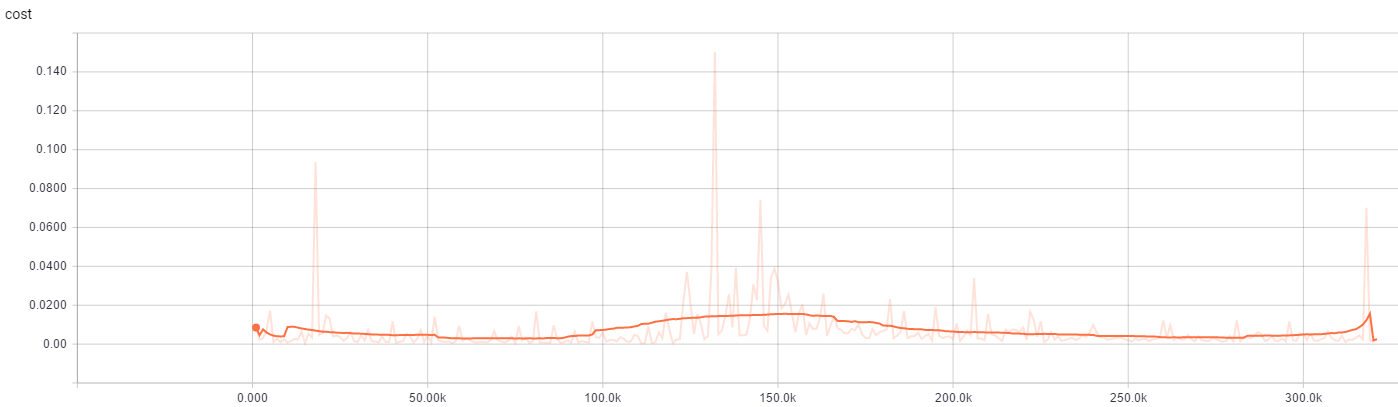FlappyBird损失变化曲线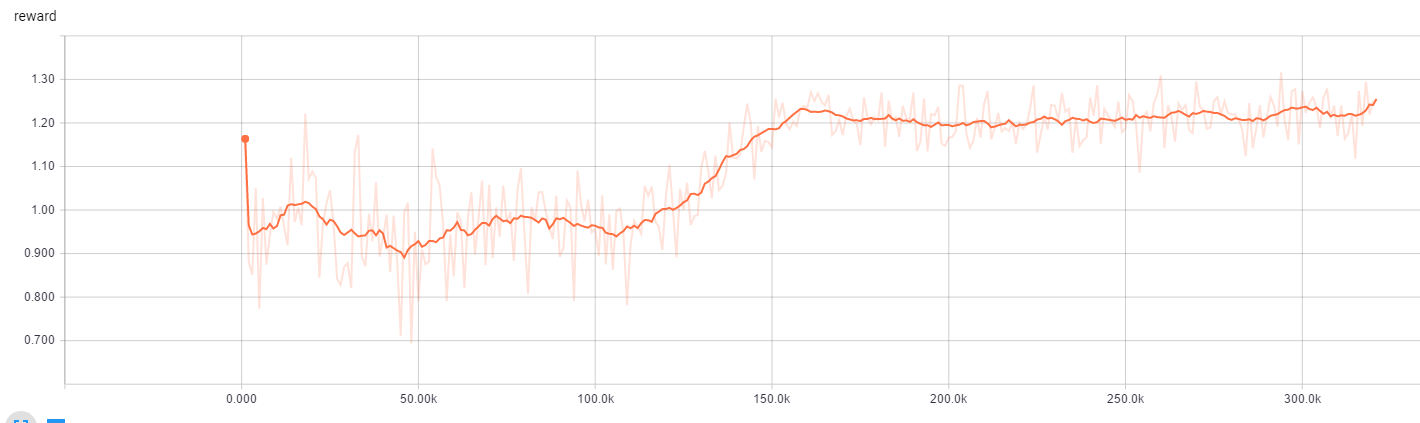FlappyBird奖励变化曲线

最后来个Gif:最后源码在这里